### Tutorial Aims:

#### 5. Synthesise information from different databases

The goal of this tutorial is to advance skills in data synthesis, particularly visualisation, manipulation, efficiently handling datasets and customising figures to make them both beautiful and informative. Here, we will focus on using packages from the `tidyverse` collection and a few extras, which together can streamline data visualisation and make your research pop out more!

## All the files you need to complete this tutorial can be downloaded from this repository. Click on `Code/Download ZIP` and unzip the folder, or clone the repository to your own GitHub account.

`R` really shines when it comes to data visualisation and with some tweaks, you can make eye-catching plots that make it easier for people to understand your science. The `ggplot2` package, part of the `tidyverse` collection of packages, as well as its many extension packages are a great tool for data visualisation, and that is the world that we will jump into over the course of this tutorial.

The `gg` in `ggplot2` stands for grammar of graphics. Writing the code for your graph is like constructing a sentence made up of different parts that logically follow from one another. In a more visual way, it means adding layers that take care of different elements of the plot. Your plotting workflow will therefore be something like creating an empty plot, adding a layer with your data points, then your measure of uncertainty, the axis labels, and so on.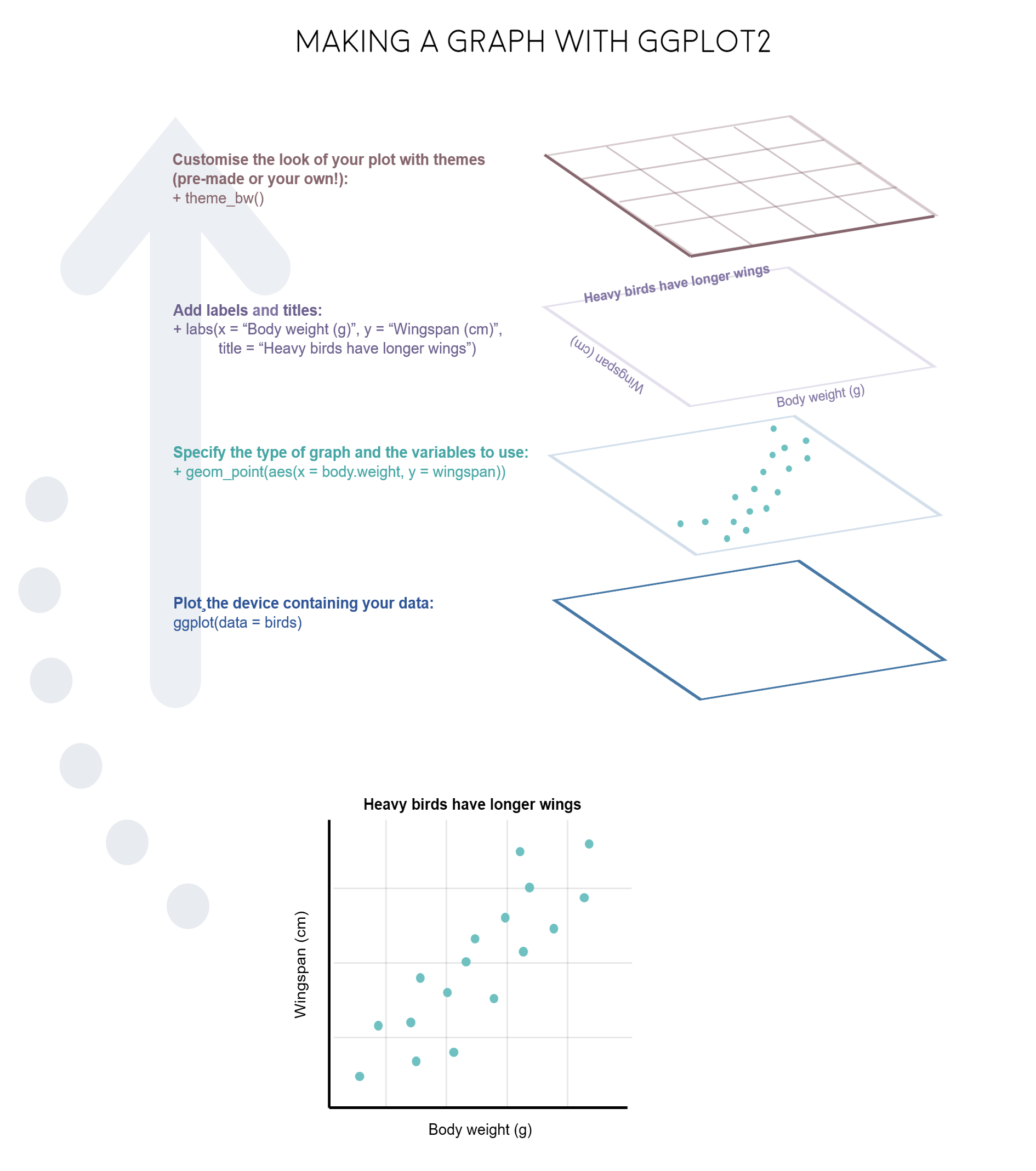Just like onions and fancy cakes, graphs in `ggplot2` have layers.

Note: Pressing enter after each “layer” of your plot (i.e. indenting it) prevents the code from being one gigantic line and makes it much easier to read.

#### Understanding `ggplot2`’s jargon

Perhaps the trickiest bit when starting out with `ggplot2` is understanding what type of elements are responsible for the contents (data) versus the container (general look) of your plot. Let’s de-mystify some of the common words you will encounter.

geom: a geometric object which defines the type of graph you are making. It reads your data in the aesthetics mapping to know which variables to use, and creates the graph accordingly. Some common types are `geom_point()`, `geom_boxplot()`, `geom_histogram()`, `geom_col()`, etc.

aes: short for aesthetics. Usually placed within a `geom_`, this is where you specify your data source and variables, AND the properties of the graph which depend on those variables. For instance, if you want all data points to be the same colour, you would define the `colour = ` argument outside the `aes()` function; if you want the data points to be coloured by a factor’s levels (e.g. by site or species), you specify the `colour = ` argument inside the `aes()`.

stat: a stat layer applies some statistical transformation to the underlying data: for instance, `stat_smooth(method = "lm")` displays a linear regression line and confidence interval ribbon on top of a scatter plot (defined with `geom_point()`).

theme: a theme is made of a set of visual parameters that control the background, borders, grid lines, axes, text size, legend position, etc. You can use pre-defined themes, create your own, or use a theme and overwrite only the elements you don’t like. Examples of elements within themes are `axis.text`, `panel.grid`, `legend.title`, and so on. You define their properties with `elements_...()` functions: `element_blank()` would return something empty (ideal for removing background colour), while `element_text(size = ..., face = ..., angle = ...)` lets you control all kinds of text properties.

Also useful to remember is that layers are added on top of each other as you progress into the code, which means that elements written later may hide or overwrite previous elements.

### Deciding on the right type of plot

A very key part of making any data visualisation is making sure that it is appropriate to your data type (e.g. discrete vs continuous), and fits your purpose, i.e. what you are trying to communicate!

Here are some common graph types, but really there is loads more, and you can visit the R Graph Galleryfor more inspiration!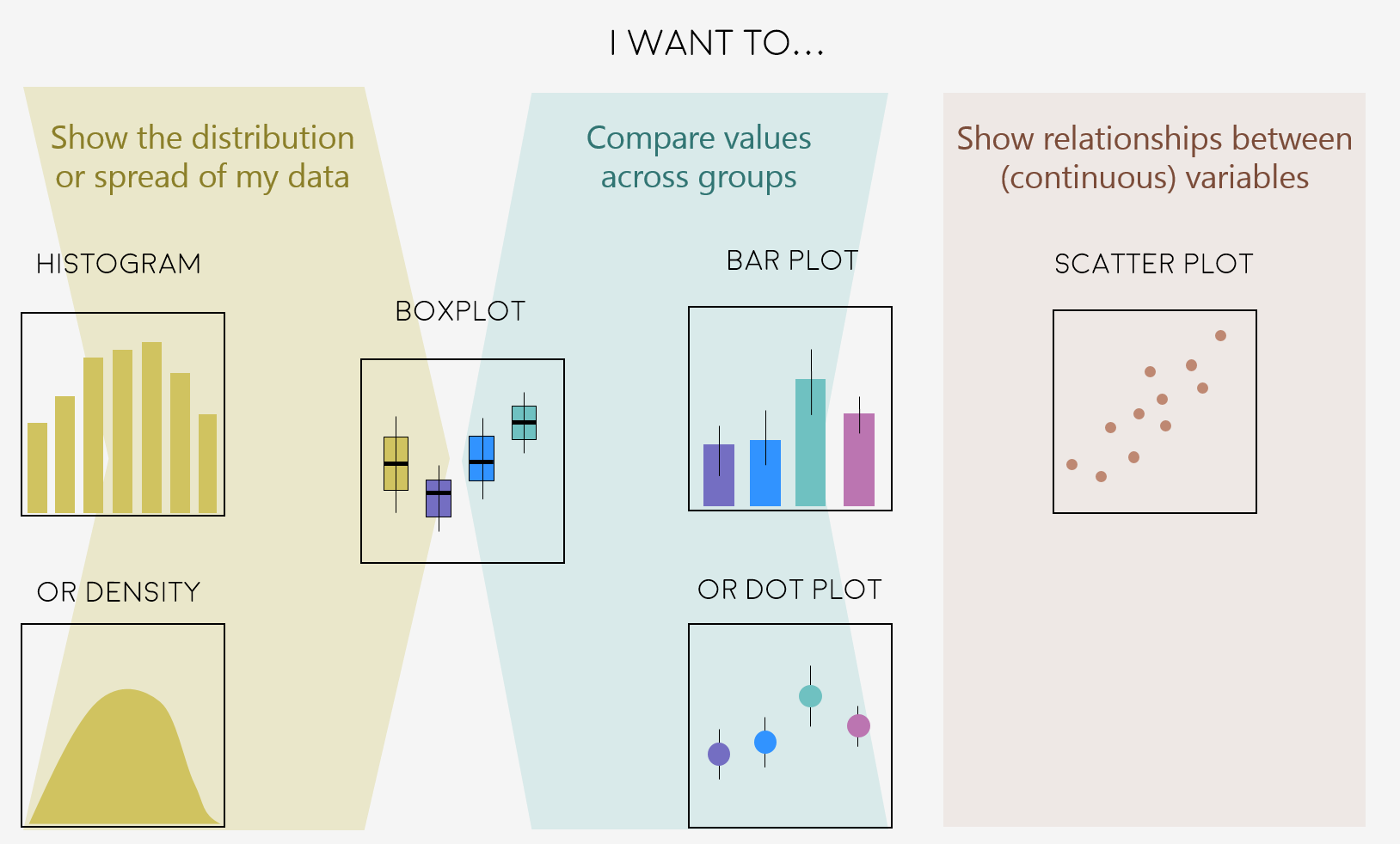Figures can change a lot the more you work on a project, and often they go on what we call a beautification journey - from a quick plot with boring or no colours to a clear and well-illustrated graph. So now that we have the data needed for the examples in this tutorial, we can start the journey.

Open `RStudio`, select `File/New File/R script` and start writing your script with the help of this tutorial. You might find it easier to have the tutorial open on half of your screen and `RStudio` on the other half, so that you can go between the two quickly.

``````# Purpose of the script
# Your name, date and email

# Your working directory, set to the folder you just downloaded from Github, e.g.:

# Libraries ----
# if you haven't installed them before, run the code install.packages("package_name")
library(tidyverse)
library(ggthemes)  # for a mapping theme

# if you have a more recent version of ggplot2, it seems to clash with the ggalt package
# installing this version of the ggalt package from GitHub solves it
# You might need to also restart your RStudio session
devtools::install_github("eliocamp/ggalt@new-coord-proj") # for custom map projections
library(ggalt)
library(ggrepel)  # for annotations
library(viridis)  # for nice colours
library(broom)  # for cleaning up models
# devtools::install_github("wilkox/treemapify")
library(treemapify)  # for making area graphs
library(wesanderson)  # for nice colours

# Data ----
# Load data - site coordinates and plant records from
# the Long Term Ecological Research Network
# https://lternet.edu and the Niwot Ridge site more specifically

``````

Managing long scripts: Lines of code pile up quickly! There is an outline feature in `RStudio` that makes long scripts more organised and easier to navigate. You can make a subsection by writing out a comment and adding four or more characters after the text, e.g. `# Section 1 ----`. If you’ve included all of the comments from the tutorial in your own script, you should already have some sections.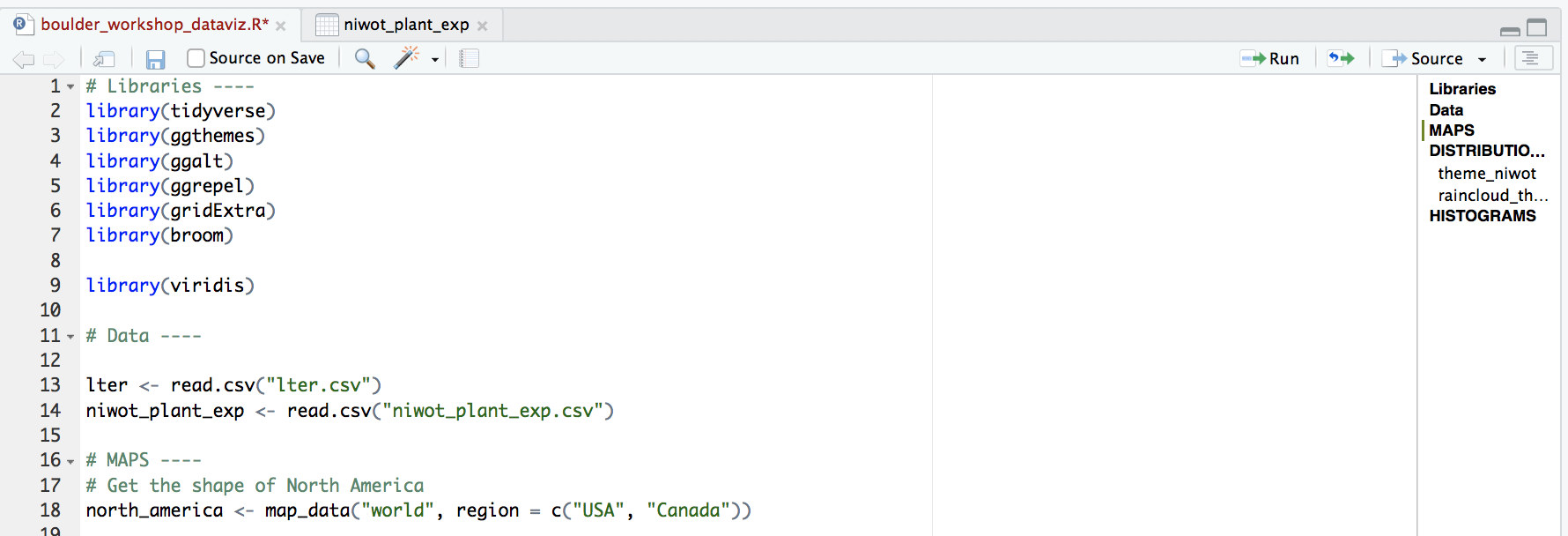An important note about graphs made using `ggplot2`: you’ll notice that throughout this tutorial, the `ggplot2` code is always surrounded by brackets. That way, we both make the graph, assign it to an object, e.g. `duration1` and we “call” the graph, so we can see it in the plot tab. If you don’t have the brackets around the code chunk, you’ll make the graph, but you won’t actually see it. Alternatively, you can “call” the graph to the plot tab by running just the line `duration1`. It’s also best to assign your graphs to objects, especially if you want to save them later, otherwise they just disappear and you’ll have to run the code again to see or save the graph.

## Make and beautify maps

Often we find ourselves needing to plot sites or species’ occurrences on a map and with `ggplot2` and a combo of a few of its companion packages, we can make nice and clear maps, with the option to choose among different map projections. Here is the journey this particular map of the sites part of the Long-Term Ecological Research Network are embarking on - small tweaks among the different steps, but ultimately the final map stands out more.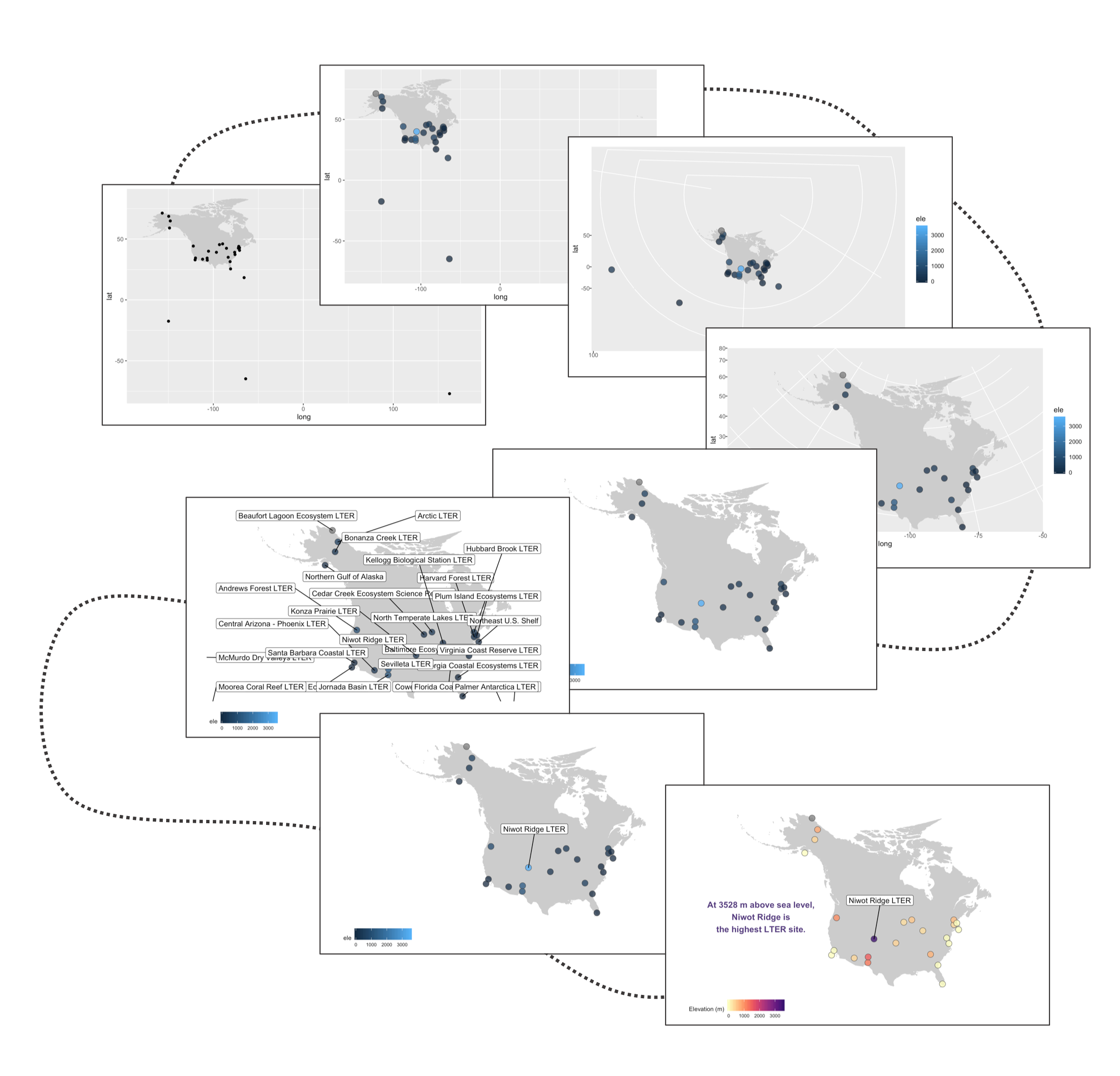``````# MAPS ----
# Get the shape of North America
north_america <- map_data("world", region = c("USA", "Canada"))

# Exclude Hawaii if you want to
north_america <- north_america[!(north_america\$subregion %in% "Hawaii"),]

# A very basic map
(lter_map1 <- ggplot() +
geom_map(map = north_america, data = north_america,
aes(long, lat, map_id = region),
color = "gray80", fill = "gray80", size = 0.3) +
# Add points for the site locations
geom_point(data = lter,
aes(x = long, y = lat)))

# You can ignore this warning message, it's cause we have forced
# specific lat and long columns onto geom_map()
# Warning: Ignoring unknown aesthetics: x, y

# if you wanted to save this (not amazing) map
# you can use ggsave()
ggsave(lter_map1, filename = "map1.png",
height = 5, width = 8)  # the units by default are in inches

# the map will be saved in your working directory
# if you have forgotten where that is, use this code to find out
getwd()
``````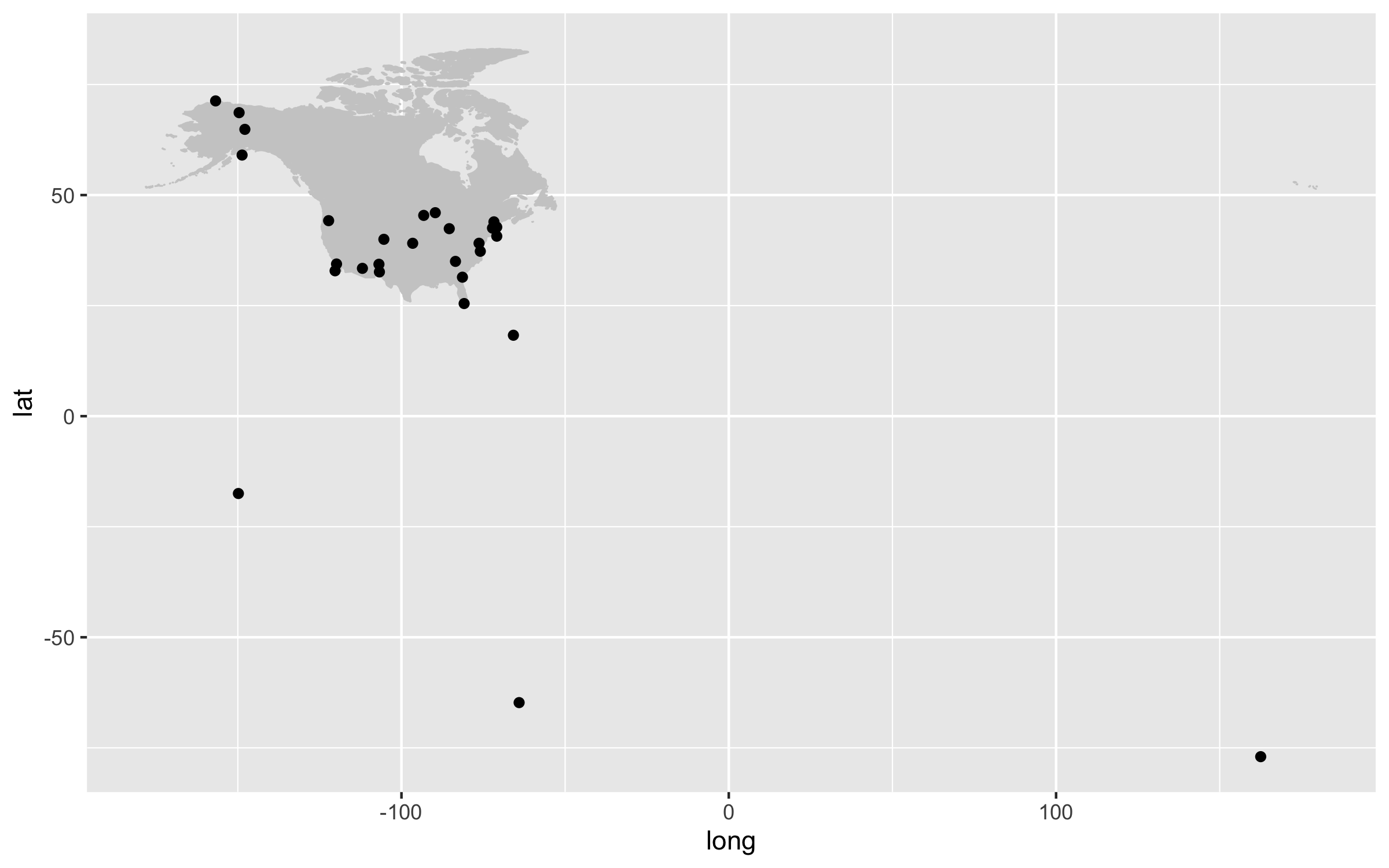Our first map does a not terrible job at visualising where the sites are, but it looks rather off and is not particularly great to look at. It’s also not communicating much information other than where the sites are. For example, we can use colours to indicate the elevation of each site.

``````(lter_map2 <- ggplot() +
geom_map(map = north_america, data = north_america,
aes(long, lat, map_id = region),
color = "gray80", fill = "gray80", size = 0.3) +
geom_point(data = lter,
aes(x = long, y = lat, fill = ele),
# when you set the fill or colour to vary depending on a variable
# you put that (e.g., fill = ele) inside the aes() call
# when you want to set a specific colour (e.g., colour = "grey30"),
# that goes outside of the aes() call
alpha = 0.8, size = 4, colour = "grey30",
shape = 21))

ggsave(lter_map2, filename = "map2.png",
height = 5, width = 8)
``````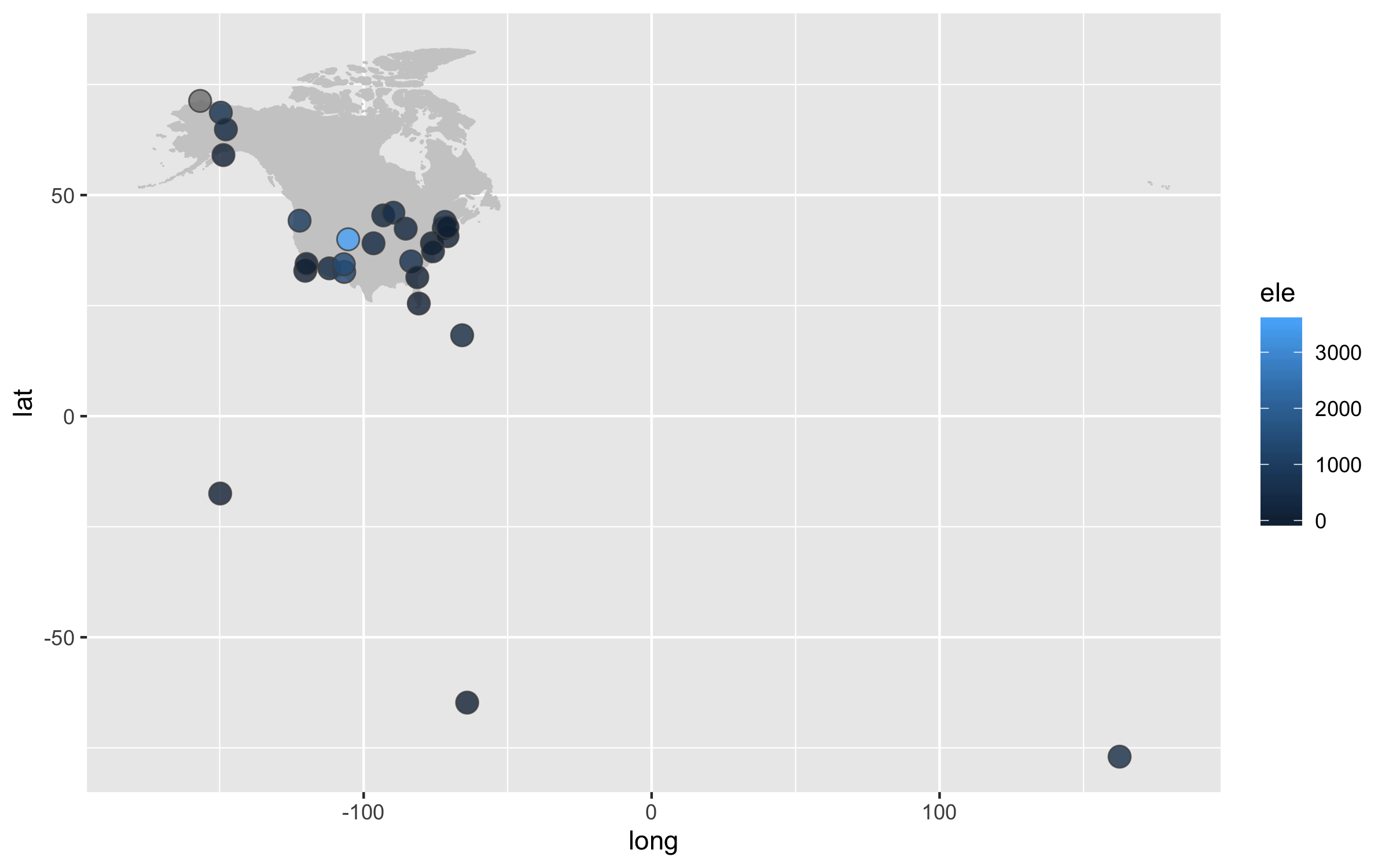Next up we can work on improving the map projection - by default we get the Mercator projection but that doesn’t represent the world very realistically. With the `ggalt` package and the `coord_proj` function, we can easily swap the default projection.

``````(lter_map3 <- ggplot() +
geom_map(map = north_america, data = north_america,
aes(long, lat, map_id = region),
color = "gray80", fill = "gray80", size = 0.3) +
# you can change the projection here
# coord_proj("+proj=wintri") +
# the wintri one above is good for the whole world, the one below for just North America
coord_proj(paste0("+proj=aea +lat_1=29.5 +lat_2=45.5 +lat_0=37.5 +lon_0=-96",
" +x_0=0 +y_0=0 +ellps=GRS80 +datum=NAD83 +units=m +no_defs")) +
geom_point(data = lter,
aes(x = long, y = lat, fill = ele),
alpha = 0.8, size = 4, colour = "grey30",
shape = 21))

# You don't need to worry about the warning messages
# that's just cause we've overwritten the default projection

ggsave(lter_map3, filename = "map3.png",
height = 5, width = 8)
``````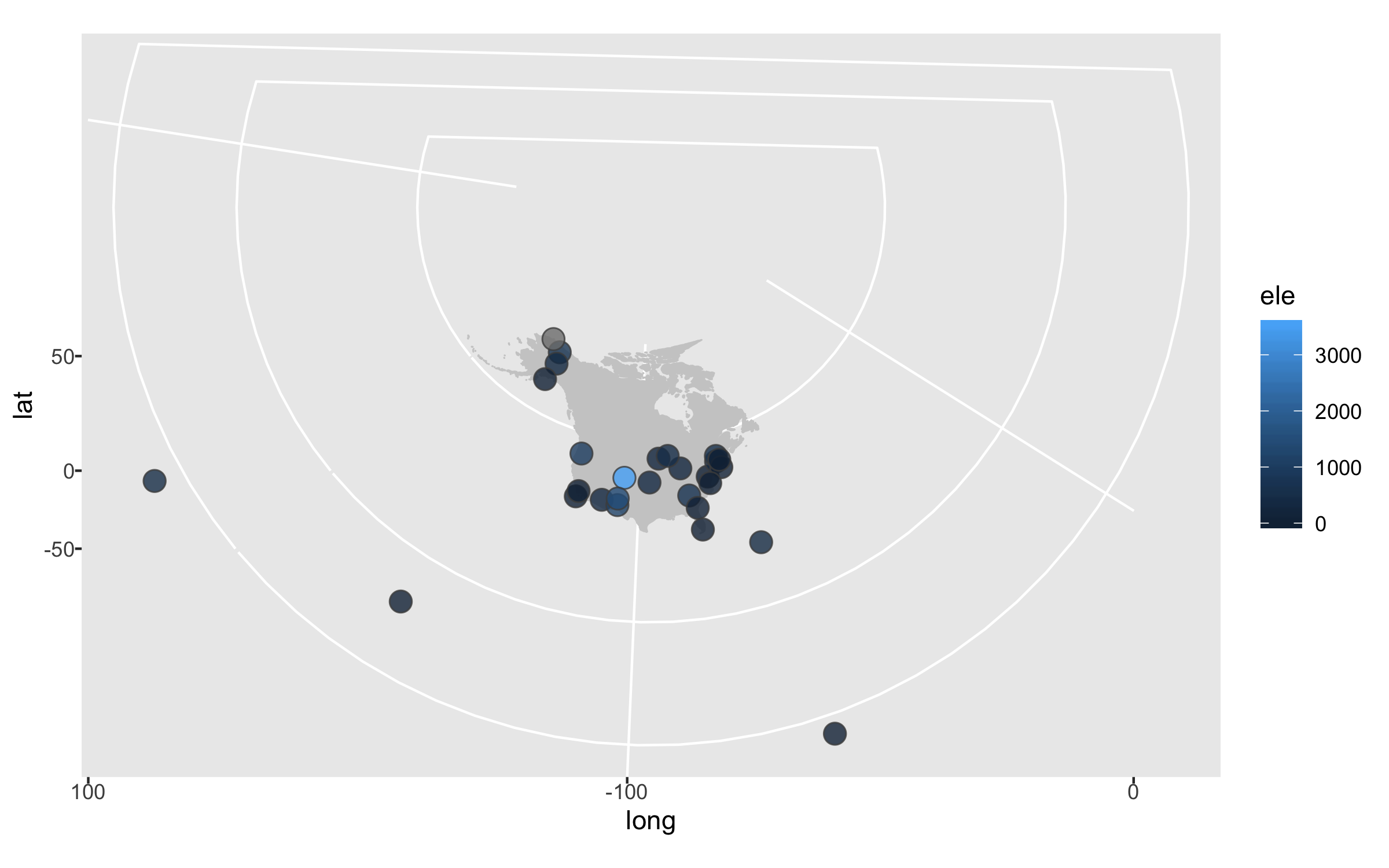The projection is better now, but because there are a few faraway sites, the map looks quite small. Since those sites are not going to be our focus, we can zoom in on the map.

``````(lter_map4 <- ggplot() +
geom_map(map = north_america, data = north_america,
aes(long, lat, map_id = region),
color = "gray80", fill = "gray80", size = 0.3) +
coord_proj(paste0("+proj=aea +lat_1=29.5 +lat_2=45.5 +lat_0=37.5 +lon_0=-96",
" +x_0=0 +y_0=0 +ellps=GRS80 +datum=NAD83 +units=m +no_defs"),
# zooming in by setting specific coordinates
ylim = c(25, 80), xlim = c(-175, -50)) +
geom_point(data = lter,
aes(x = long, y = lat, fill = ele),
alpha = 0.8, size = 4, colour = "grey30",
shape = 21))

ggsave(lter_map4, filename = "map4.png",
height = 5, width = 8)
``````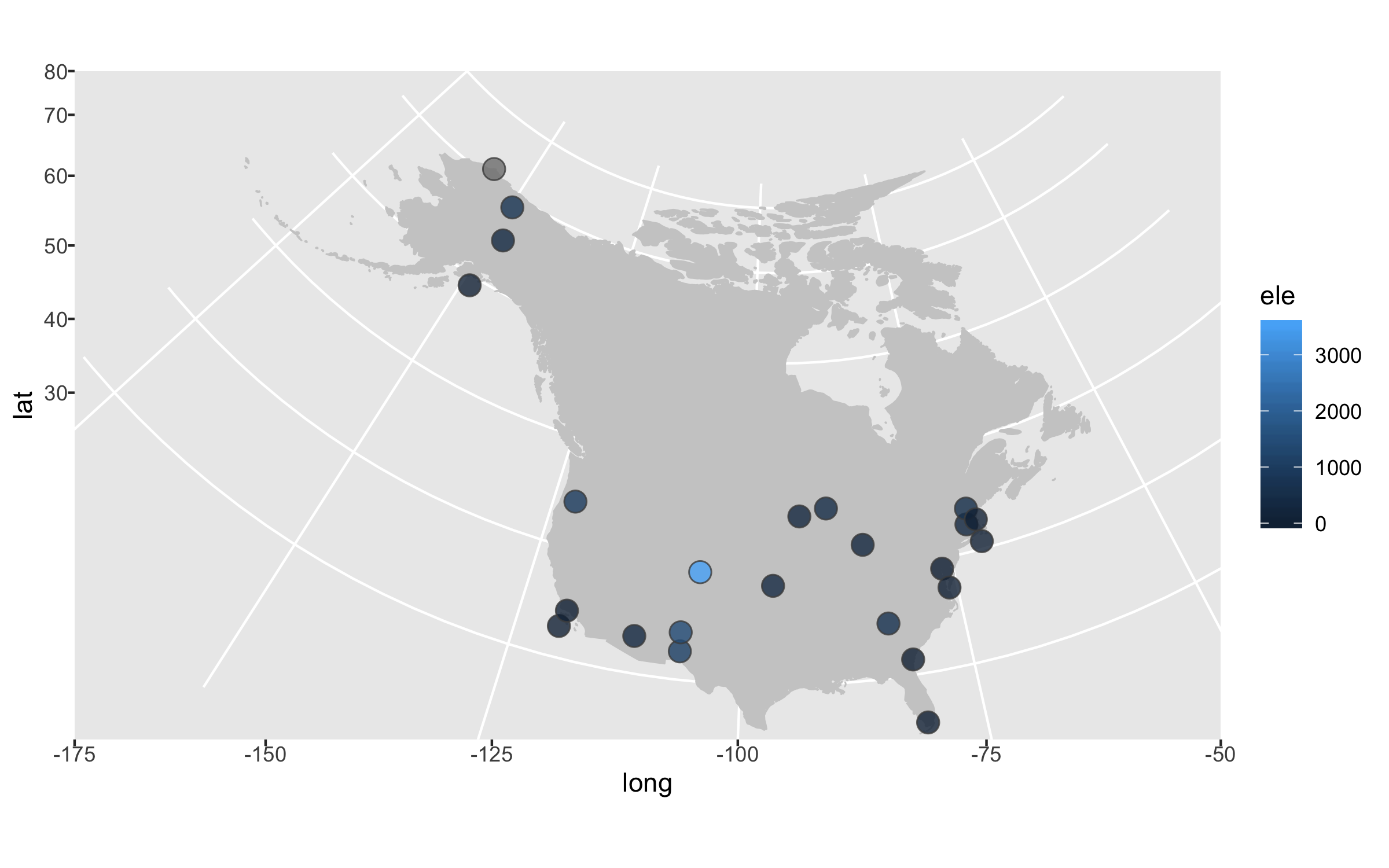Next up we can declutter a bit - we don’t really need that grey background and people know that on a map you have latitude and longitude as the axes.

``````(lter_map5 <- ggplot() +
geom_map(map = north_america, data = north_america,
aes(long, lat, map_id = region),
color = "gray80", fill = "gray80", size = 0.3) +
coord_proj(paste0("+proj=aea +lat_1=29.5 +lat_2=45.5 +lat_0=37.5 +lon_0=-96",
" +x_0=0 +y_0=0 +ellps=GRS80 +datum=NAD83 +units=m +no_defs"),
ylim = c(25, 80), xlim = c(-175, -50)) +
geom_point(data = lter,
aes(x = long, y = lat, fill = ele),
alpha = 0.8, size = 4, colour = "grey30",
shape = 21) +
# Adding a clean map theme
theme_map() +
# Putting the legend at the bottom
theme(legend.position = "bottom"))

ggsave(lter_map5, filename = "map5.png",
height = 5, width = 8)
``````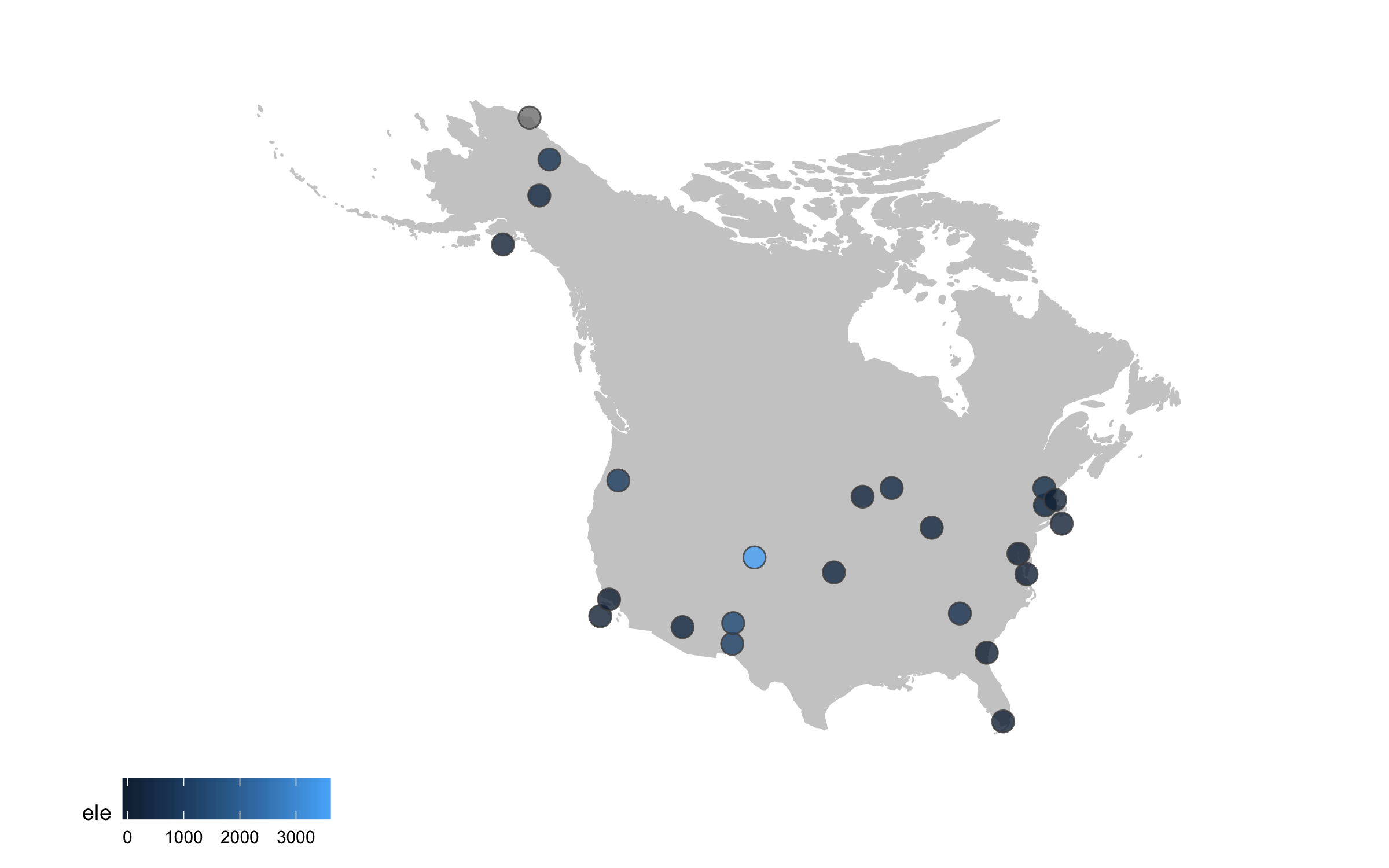Sometimes we want to annotate points and communicate what’s where - the `ggrepel` package is very useful in such cases.

``````(lter_map6 <- ggplot() +
geom_map(map = north_america, data = north_america,
aes(long, lat, map_id = region),
color = "gray80", fill = "gray80", size = 0.3) +
coord_proj(paste0("+proj=aea +lat_1=29.5 +lat_2=45.5 +lat_0=37.5 +lon_0=-96",
" +x_0=0 +y_0=0 +ellps=GRS80 +datum=NAD83 +units=m +no_defs"),
ylim = c(25, 80), xlim = c(-175, -50)) +
geom_point(data = lter,
aes(x = long, y = lat, fill = ele),
alpha = 0.8, size = 4, colour = "grey30",
shape = 21) +
theme_map() +
theme(legend.position = "bottom") +
# Adding point annotations with the site name
geom_label_repel(data = lter,
aes(x = long, y = lat,
label = site),
# Setting the positions of the labels
box.padding = 1, size = 4, nudge_x = 1, nudge_y = 1))

ggsave(lter_map6, filename = "map6.png",
height = 5, width = 8)
``````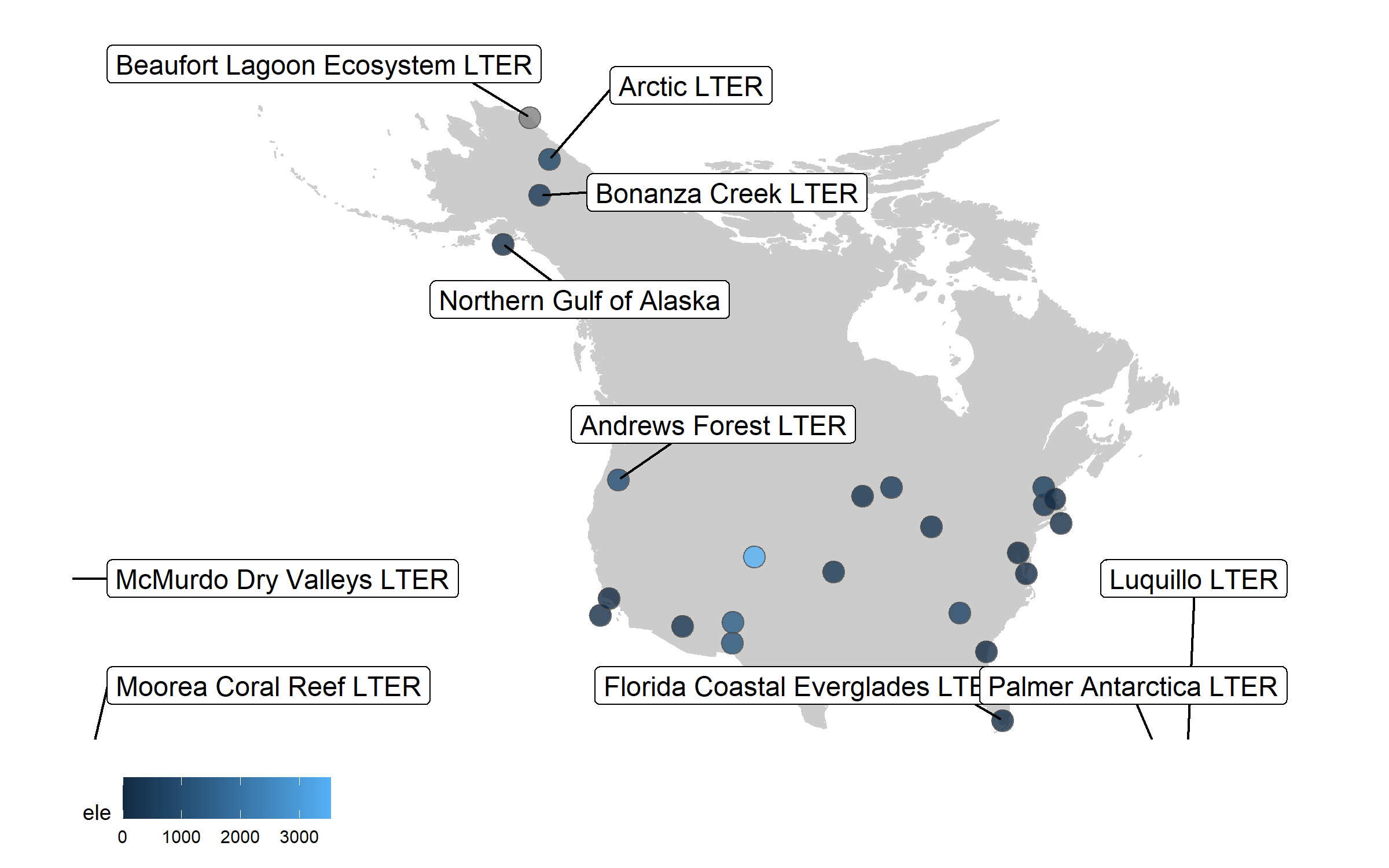Well, we slightly overdid it with the labels, so we got a warning that there are too many labels and a map where they don’t overlap a lot couldn’t be constructed. (Depending on your version of packages, you may instead get a map with all the labels, but with too many of them overlapping). But where annotations really shine is in drawing attention to a specific point or data record. So we can add a label just for one of the sites, Niwot Ridge, from where the plant data for the rest of the tutorial comes.

``````(lter_map7 <- ggplot() +
geom_map(map = north_america, data = north_america,
aes(long, lat, map_id = region),
color = "gray80", fill = "gray80", size = 0.3) +
coord_proj(paste0("+proj=aea +lat_1=29.5 +lat_2=45.5 +lat_0=37.5 +lon_0=-96",
" +x_0=0 +y_0=0 +ellps=GRS80 +datum=NAD83 +units=m +no_defs"),
ylim = c(25, 80), xlim = c(-175, -50)) +
geom_point(data = lter,
aes(x = long, y = lat, fill = ele),
alpha = 0.8, size = 4, colour = "grey30",
shape = 21) +
theme_map() +
theme(legend.position = "bottom") +
geom_label_repel(data = subset(lter, ele > 2000),
aes(x = long, y = lat,
label = site),
box.padding = 1, size = 4, nudge_x = 1, nudge_y = 12))

ggsave(lter_map7, filename = "map7.png",
height = 5, width = 8)
``````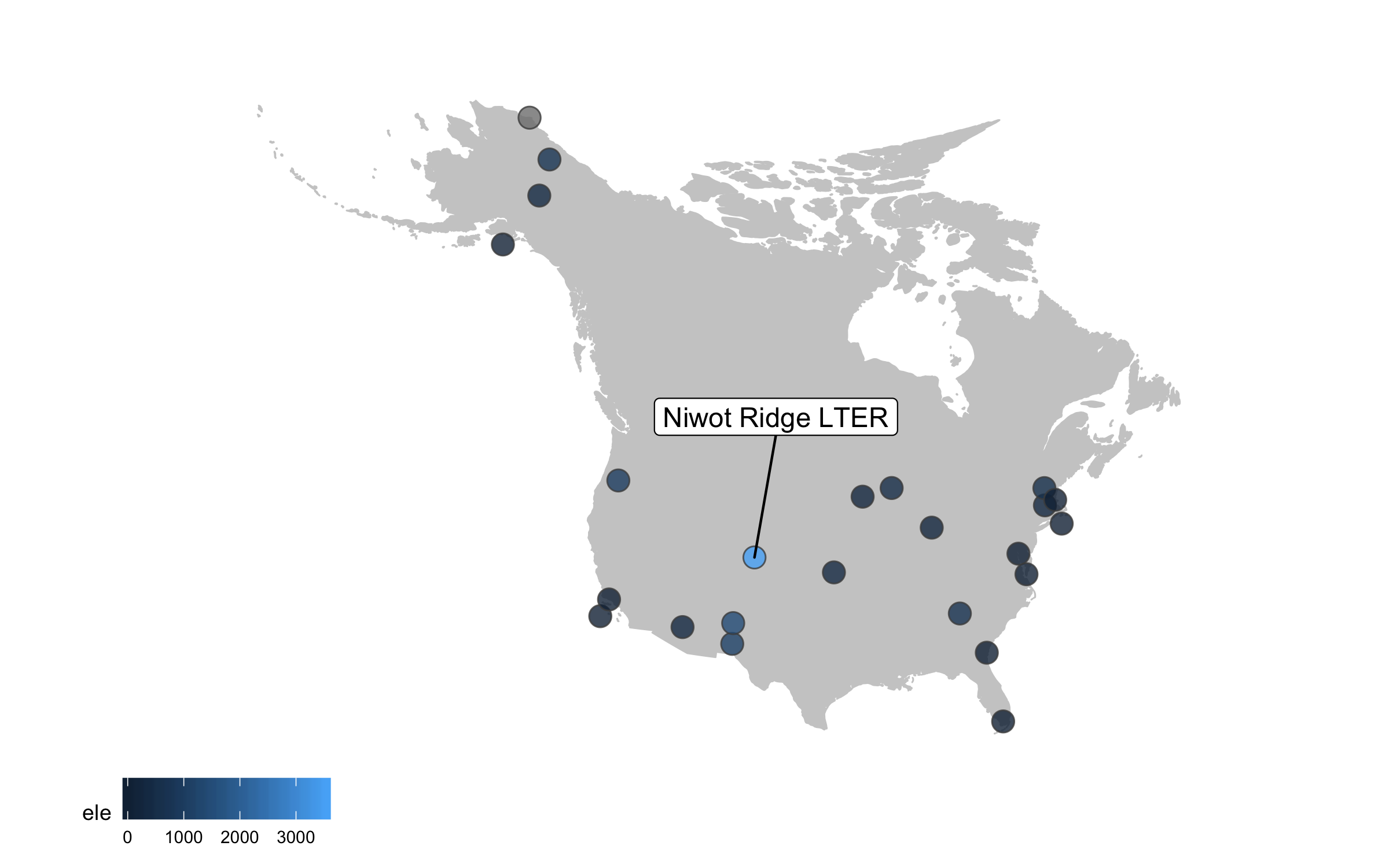This is looking better, but the colours are not very exciting. Depending on the purpose of the map and where it’s going (e.g., presentation, manuscript, a science communication piece), we can also add some text with an interesting fact about the site.

``````(lter_map8 <- ggplot() +
geom_map(map = north_america, data = north_america,
aes(long, lat, map_id = region),
color = "gray80", fill = "gray80", size = 0.3) +
coord_proj(paste0("+proj=aea +lat_1=29.5 +lat_2=45.5 +lat_0=37.5 +lon_0=-96",
" +x_0=0 +y_0=0 +ellps=GRS80 +datum=NAD83 +units=m +no_defs"),
ylim = c(25, 80), xlim = c(-175, -50)) +
geom_point(data = lter,
aes(x = long, y = lat, fill = ele),
alpha = 0.8, size = 4, colour = "grey30",
shape = 21) +
theme_map() +
theme(legend.position = "bottom") +
geom_label_repel(data = subset(lter, ele > 2000),
aes(x = long, y = lat,
label = site),
box.padding = 1, size = 4, nudge_x = 1, nudge_y = 12) +
labs(fill = "Elevation (m)") +
annotate("text", x = -150, y = 35, colour = "#553c7f",
label = "At 3528 m above sea level,\nNiwot Ridge is\nthe highest LTER site.",
size = 4.5, fontface = "bold") +
scale_fill_viridis(option = "magma", direction = -1, begin = 0.2))

ggsave(lter_map8, filename = "map8.png",
height = 5, width = 8)
``````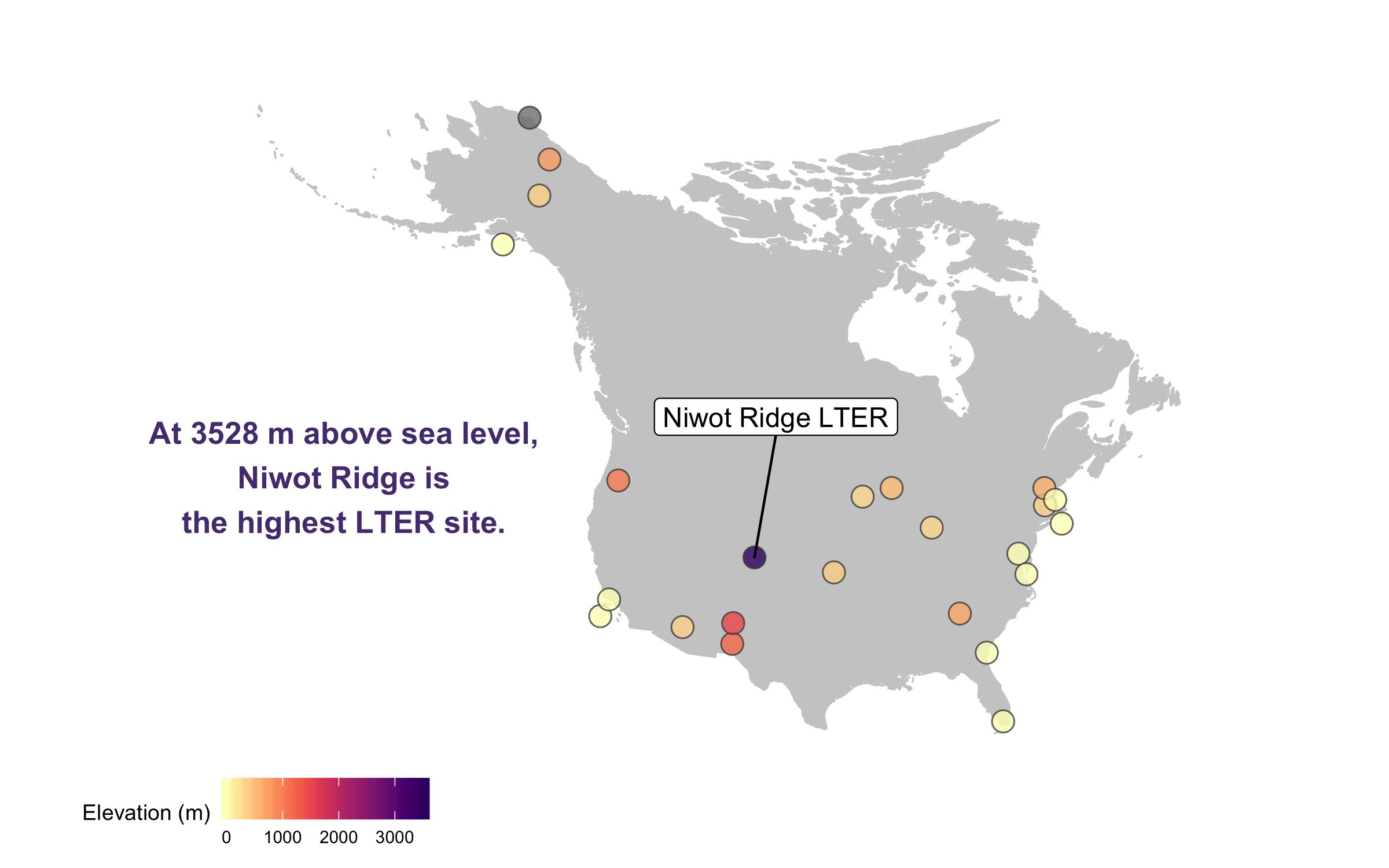There goes our map! Hard to say our “finished” map, because figures evolve a lot, but for now we’ll leave the map here and move onto distributions - a great way to communicate the whole spectrum of variance in your dataset!

## Visualise distributions (and make them rain data with raincloud plots)

Behind every mean, there is a distribution, and that distribution has a story tell, if only we let it! Visualising distributions is a very useful way to communicate patterns in your data in a more transparent way than just a mean and its error.

Violin plots (the fatter the violin at a given value, the more data points there) are pretty and sound poetic, but we can customise them to make their messages pop out more. Thus the beautification journey begins again.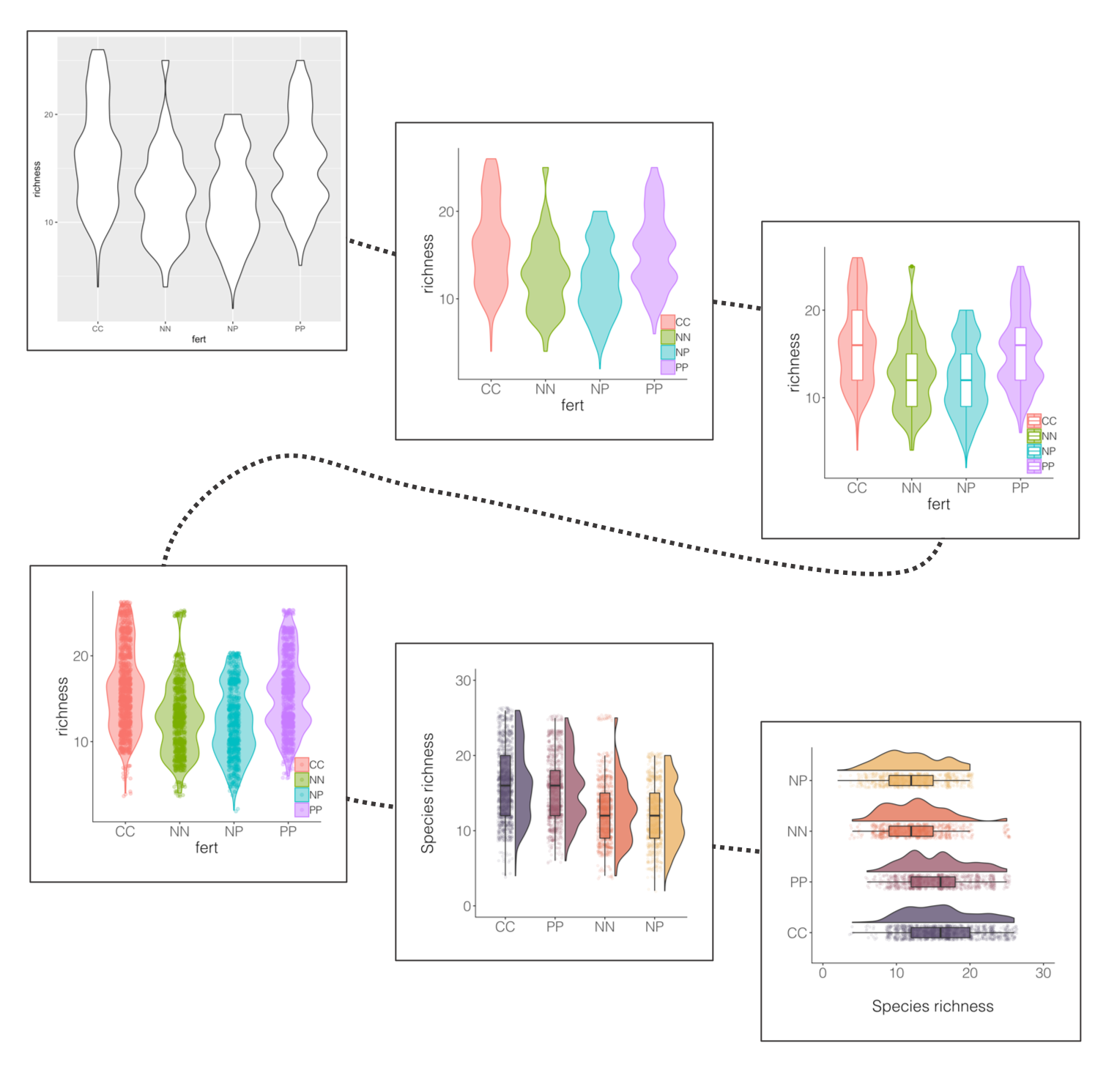If you’ve ever tried to perfect your `ggplot2` graphs, you might have noticed that the lines starting with `theme()` quickly pile up: you adjust the font size of the axes and the labels, the position of the title, the background colour of the plot, you remove the grid lines in the background, etc. And then you have to do the same for the next plot, which really increases the amount of code you use. Here is a simple solution: create a customised theme that combines all the `theme()` elements you want and apply it to your graphs to make things easier and increase consistency. You can include as many elements in your theme as you want, as long as they don’t contradict one another and then when you apply your theme to a graph, only the relevant elements will be considered.

``````# DISTRIBUTIONS ----
# Setting a custom ggplot2 function
# This function makes a pretty ggplot theme
# This function takes no arguments
# meaning that you always have just niwot_theme() and not niwot_theme(something else here)

theme_niwot <- function(){
theme_bw() +
theme(text = element_text(family = "Helvetica Light"),
axis.text = element_text(size = 16),
axis.title = element_text(size = 18),
axis.line.x = element_line(color="black"),
axis.line.y = element_line(color="black"),
panel.border = element_blank(),
panel.grid.major.x = element_blank(),
panel.grid.minor.x = element_blank(),
panel.grid.minor.y = element_blank(),
panel.grid.major.y = element_blank(),
plot.margin = unit(c(1, 1, 1, 1), units = , "cm"),
plot.title = element_text(size = 18, vjust = 1, hjust = 0),
legend.text = element_text(size = 12),
legend.title = element_blank(),
legend.position = c(0.95, 0.15),
legend.key = element_blank(),
legend.background = element_rect(color = "black",
fill = "transparent",
size = 2, linetype = "blank"))
}

``````

First up, we should decide on a variable whose distribution we will show. The data we are working with represent plant species and how often they were recorded at a fertilisation experiment at the Niwot Ridge LTER site. There are multiple plots per fertilisation treatment and they were monitored in several years, so one thing we can calculate from these data is the number of species per plot per year.

A data manipulation tip: Pipes (%>%) are great for streamlining data analysis. If you haven’t used them before, you can find an intro in our tutorial here. A useful way to familiariase yourself with what the pipe does at each step is to “break” the pipe and check out what the resulting object looks like if you’ve only ran the code up to a certain point. You can do that by just select the relevant bit of code and running only that, but remember you have to exclude the piping operator at the end of the line, so e.g. you select up to `niwot_richness <- niwot_plant_exp %>% group_by(plot_num, year)` and not the whole `niwot_richness <- niwot_plant_exp %>% group_by(plot_num, year) %>%`.

Running pipes sequentially line by line also comes in handy when there is an error in your pipe and you don’t know which part exactly introduces the error.

Grouping by a certain variable is probably one of the most commonly used functions from the `tidyverse` (e.g., in our case we group by year and plot to calculate species richness for every combo of those two grouping variables), but remember to ungroup afterwards as if you forget, the grouping remains even if you don’t “see” it and that might later on lead to some unintended consequences.

``````# Calculate species richness per plot per year
niwot_richness <- niwot_plant_exp %>% group_by(plot_num, year) %>%
mutate(richness = length(unique(USDA_Scientific_Name))) %>% ungroup()

``````

Now that we have calculated the species richness, we can visualise how it varies across fertilisation treatments.

``````(distributions1 <- ggplot(niwot_richness, aes(x = fert, y = richness)) +
geom_violin())

ggsave(distributions1, filename = "distributions1.png",
height = 5, width = 5)

``````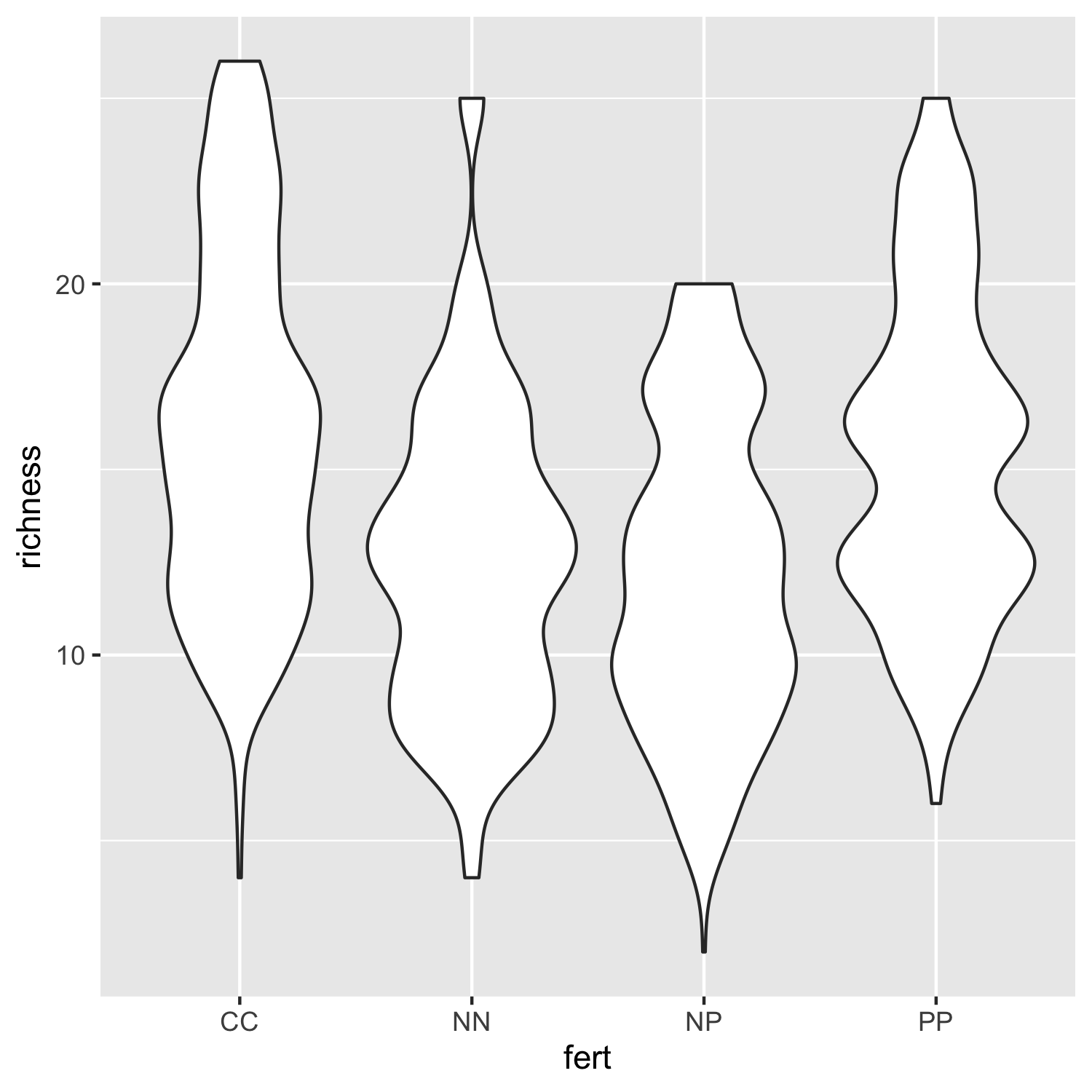Not that inspiring, but a useful first look at the data distributions. We can bring some colour in to make it more exciting and also add our custom theme so that the plot is clearer.

``````(distributions2 <- ggplot(niwot_richness, aes(x = fert, y = richness)) +
geom_violin(aes(fill = fert, colour = fert), alpha = 0.5) +
# alpha controls the opacity
theme_niwot())

ggsave(distributions2, filename = "distributions2.png",
height = 5, width = 5)
``````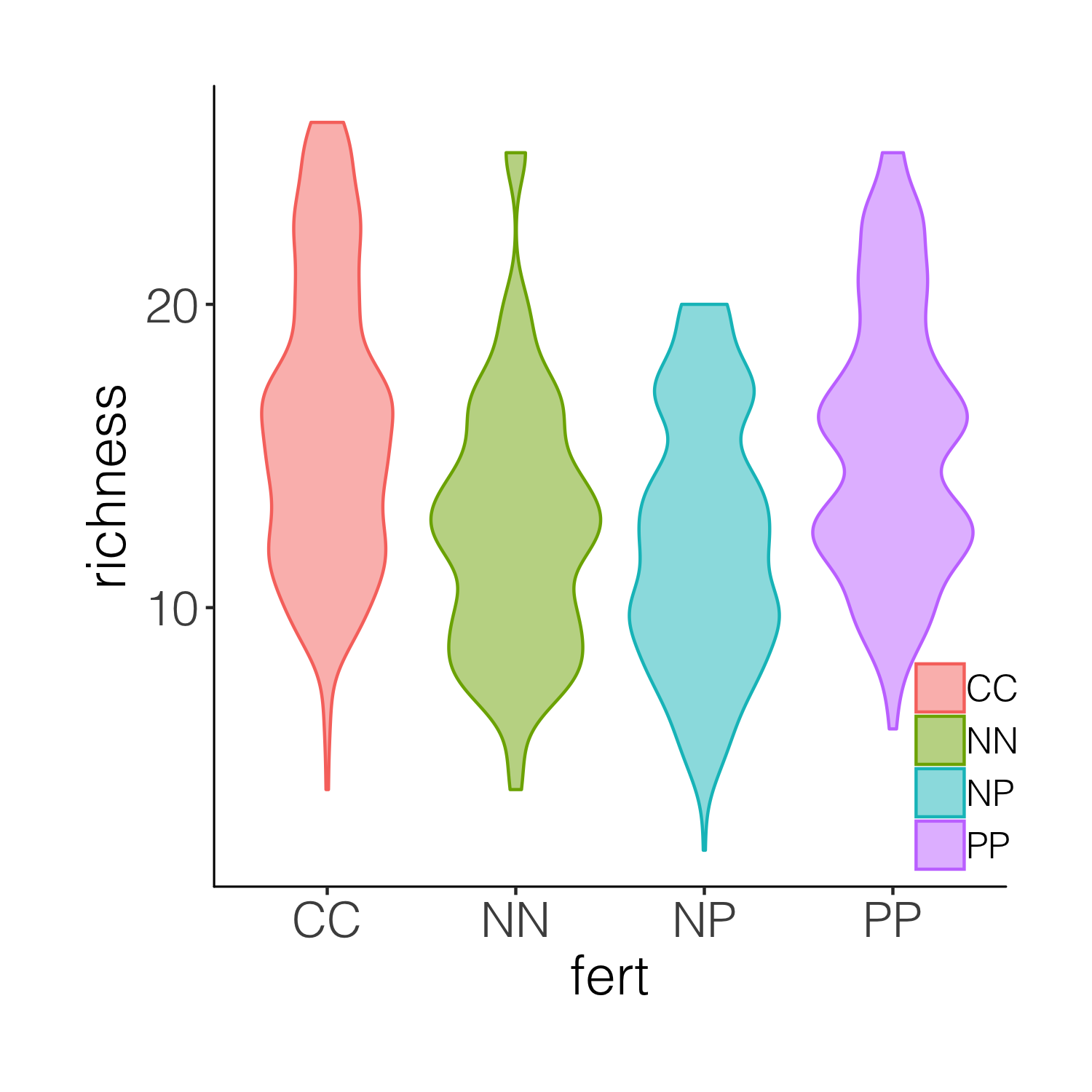You may get a warning that the font in the theme is not available on your computer. If that happens, go back to the code chunk constructing `theme_niwot()` and remove the font line `text = element_text(family = "Helvetica Light"),` or replace the font with another one you have available. Then, remember to re-run that code chunk to update the function before proceeding.

This graph is better, but it’s still taxing on a reader or observer of the graph to figure out, for example, where is the mean in each cateogry. Thus we can overlay the violins with box plots.

``````(distributions3 <- ggplot(niwot_richness, aes(x = fert, y = richness)) +
geom_violin(aes(fill = fert, colour = fert), alpha = 0.5) +
geom_boxplot(aes(colour = fert), width = 0.2) +
theme_niwot())

ggsave(distributions3, filename = "distributions3.png",
height = 5, width = 5)
``````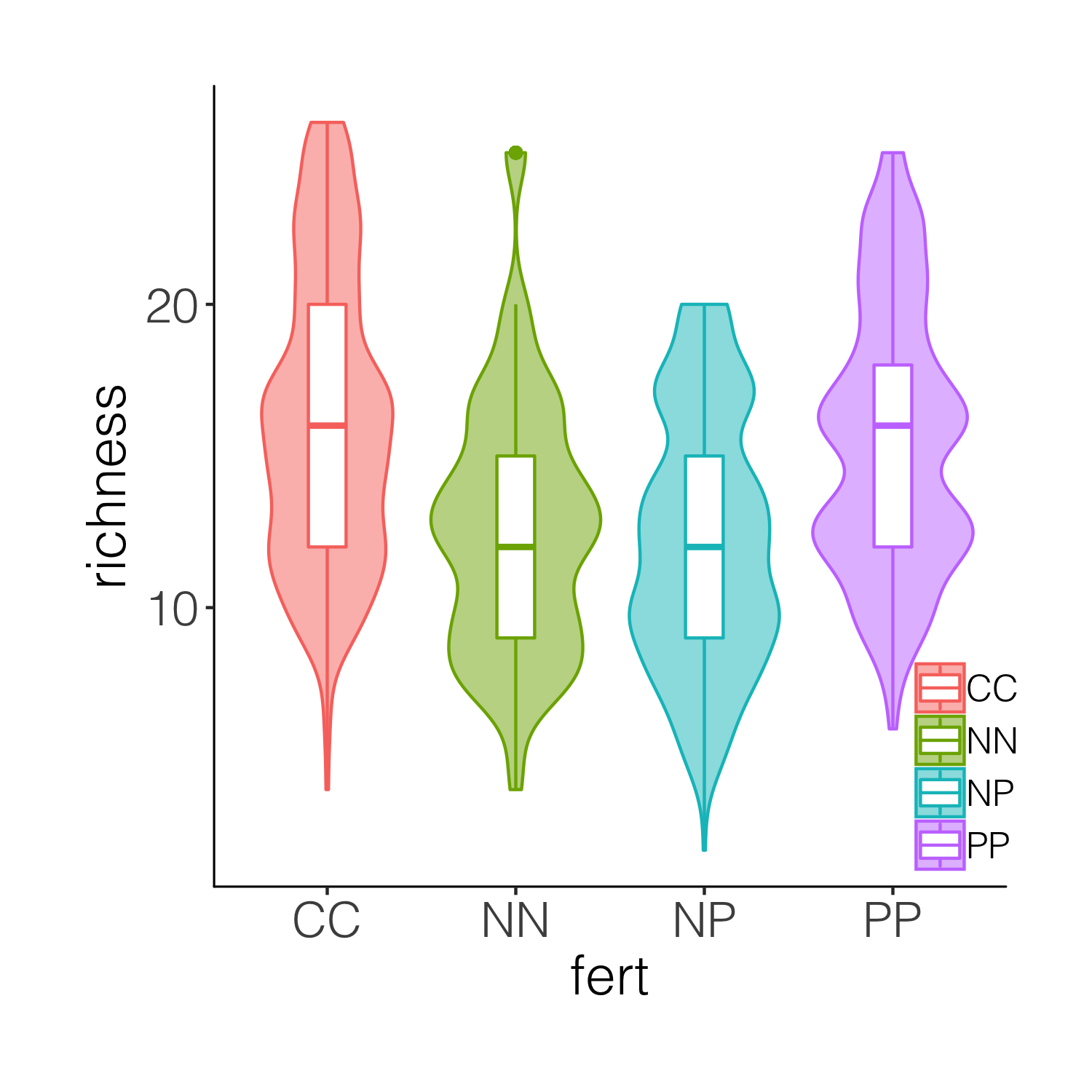While the boxplots do add some more information on the plot, we still don’t know exactly where the data points are, and the smoothing function for violins can sometimes hide the real value of a given variable. So intead of a boxplot, we can add the actual data points.

``````(distributions4 <- ggplot(niwot_richness, aes(x = fert, y = richness)) +
geom_violin(aes(fill = fert, colour = fert), alpha = 0.5) +
geom_jitter(aes(colour = fert), position = position_jitter(0.1),
alpha = 0.3) +
theme_niwot())

ggsave(distributions4, filename = "distributions4.png",
height = 5, width = 5)
``````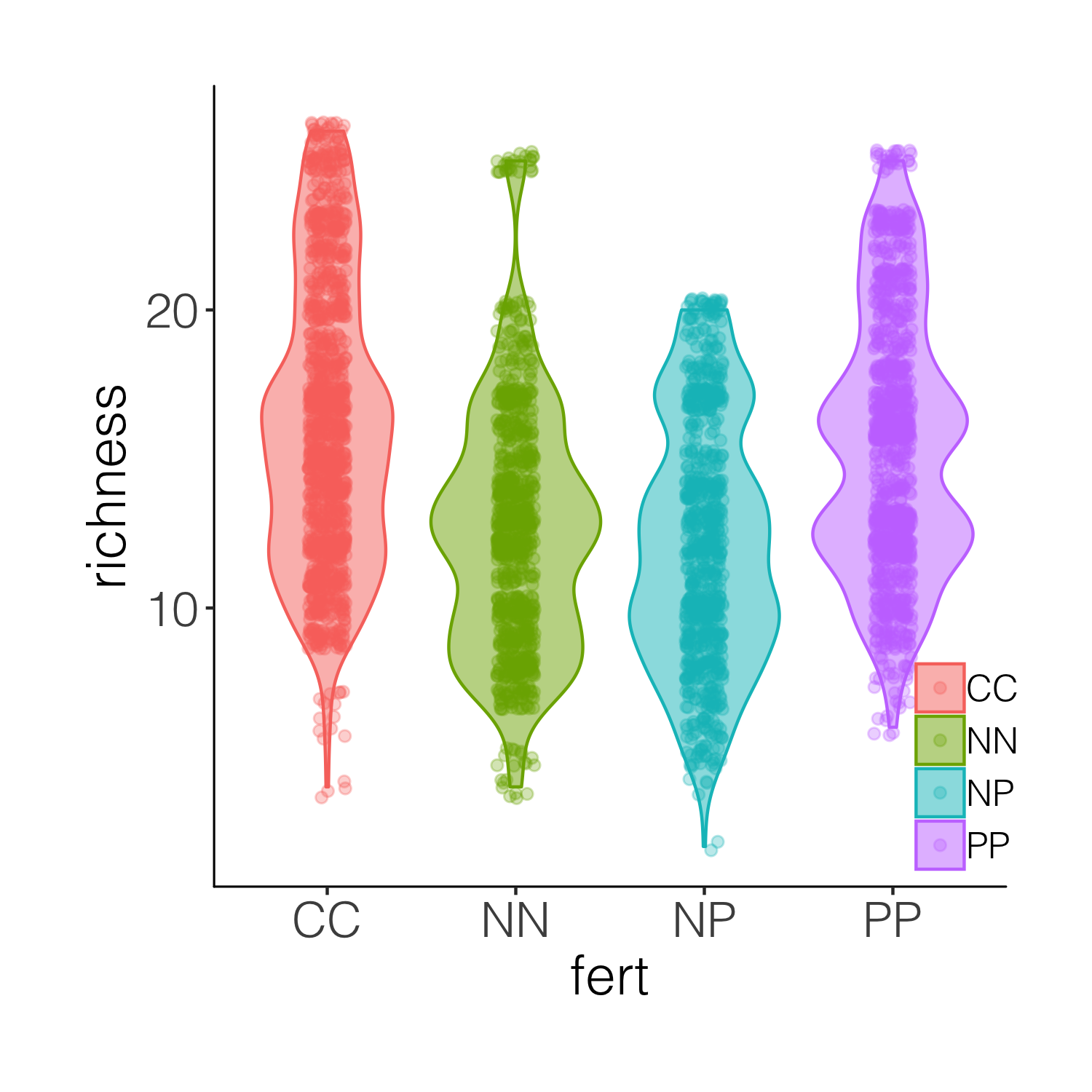A bit busy! While it’s nice to see the real data, the points are rather hard to tell apart when they are on top of the violins. And this is where raincloud plots come in! They combine a distribution with the real data points as well as a boxplot.

``````
# We will use a function by Ben Marwick
# This code loads the function in the working environment
source("https://gist.githubusercontent.com/benmarwick/2a1bb0133ff568cbe28d/raw/fb53bd97121f7f9ce947837ef1a4c65a73bffb3f/geom_flat_violin.R")

# Now we can make the plot!
(distributions5 <-
ggplot(data = niwot_richness,
aes(x = reorder(fert, desc(richness)), y = richness, fill = fert)) +
# The half violins
geom_flat_violin(position = position_nudge(x = 0.2, y = 0), alpha = 0.8) +
# The points
geom_point(aes(y = richness, color = fert),
position = position_jitter(width = 0.15), size = 1, alpha = 0.1) +
# The boxplots
geom_boxplot(width = 0.2, outlier.shape = NA, alpha = 0.8) +
# \n adds a new line which creates some space between the axis and axis title
labs(y = "Species richness\n", x = NULL) +
# Removing legends
guides(fill = FALSE, color = FALSE) +
# Setting the limits of the y axis
scale_y_continuous(limits = c(0, 30)) +
# Picking nicer colours
scale_fill_manual(values = c("#5A4A6F", "#E47250",  "#EBB261", "#9D5A6C")) +
scale_colour_manual(values = c("#5A4A6F", "#E47250",  "#EBB261", "#9D5A6C")) +
theme_niwot())

ggsave(distributions5, filename = "distributions5.png",
height = 5, width = 5)
``````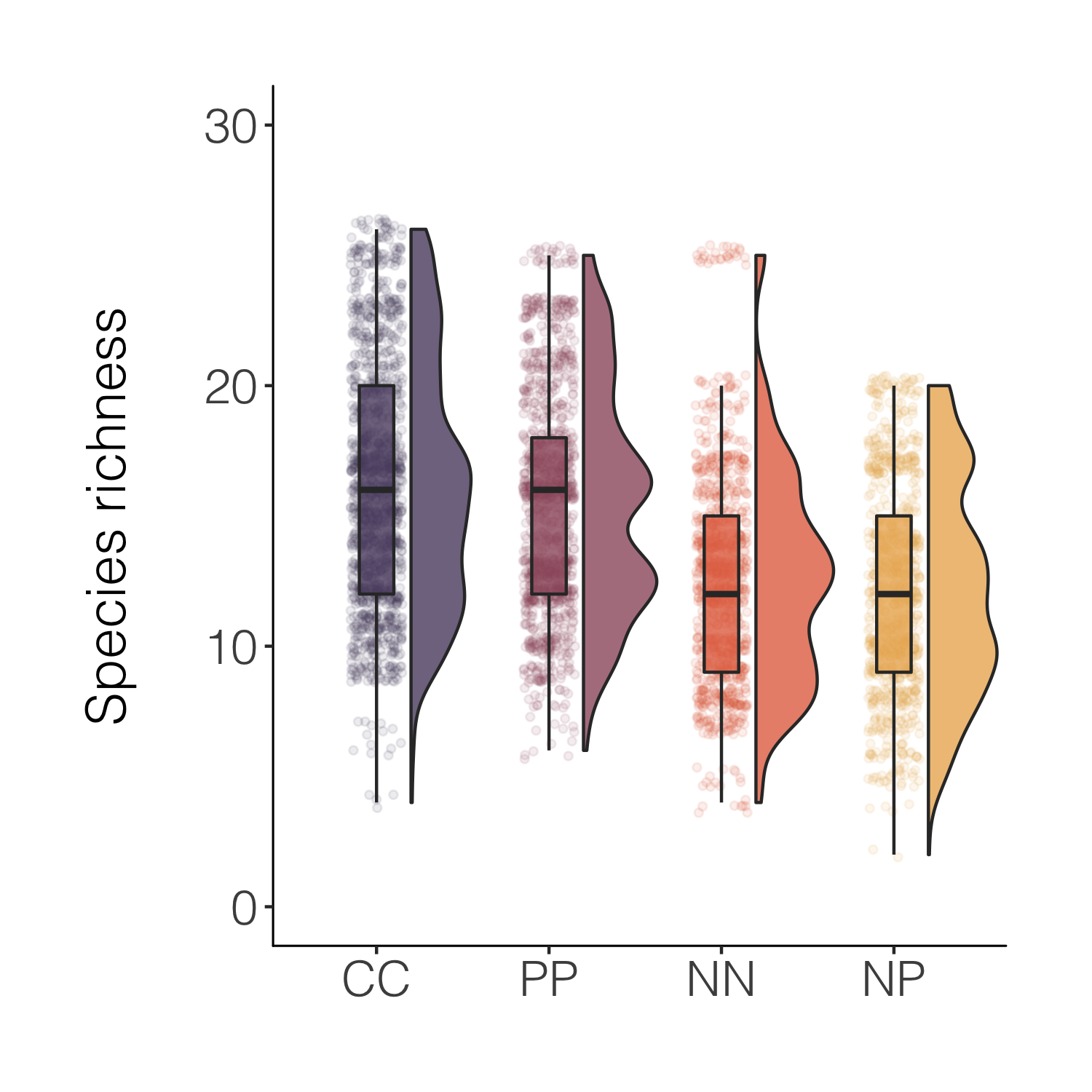That’s nicer and the combo of the different kinds of plots makes it easy to see both the distribution as well as things like the mean. For a full raincloud plot experience, we can flip the x and y axis.

``````(distributions6 <-
ggplot(data = niwot_richness,
aes(x = reorder(fert, desc(richness)), y = richness, fill = fert)) +
geom_flat_violin(position = position_nudge(x = 0.2, y = 0), alpha = 0.8) +
geom_point(aes(y = richness, color = fert),
position = position_jitter(width = 0.15), size = 1, alpha = 0.1) +
geom_boxplot(width = 0.2, outlier.shape = NA, alpha = 0.8) +
labs(y = "\nSpecies richness", x = NULL) +
guides(fill = FALSE, color = FALSE) +
scale_y_continuous(limits = c(0, 30)) +
scale_fill_manual(values = c("#5A4A6F", "#E47250",  "#EBB261", "#9D5A6C")) +
scale_colour_manual(values = c("#5A4A6F", "#E47250",  "#EBB261", "#9D5A6C")) +
coord_flip() +
theme_niwot())

ggsave(distributions6, filename = "distributions6.png",
height = 5, width = 5)
``````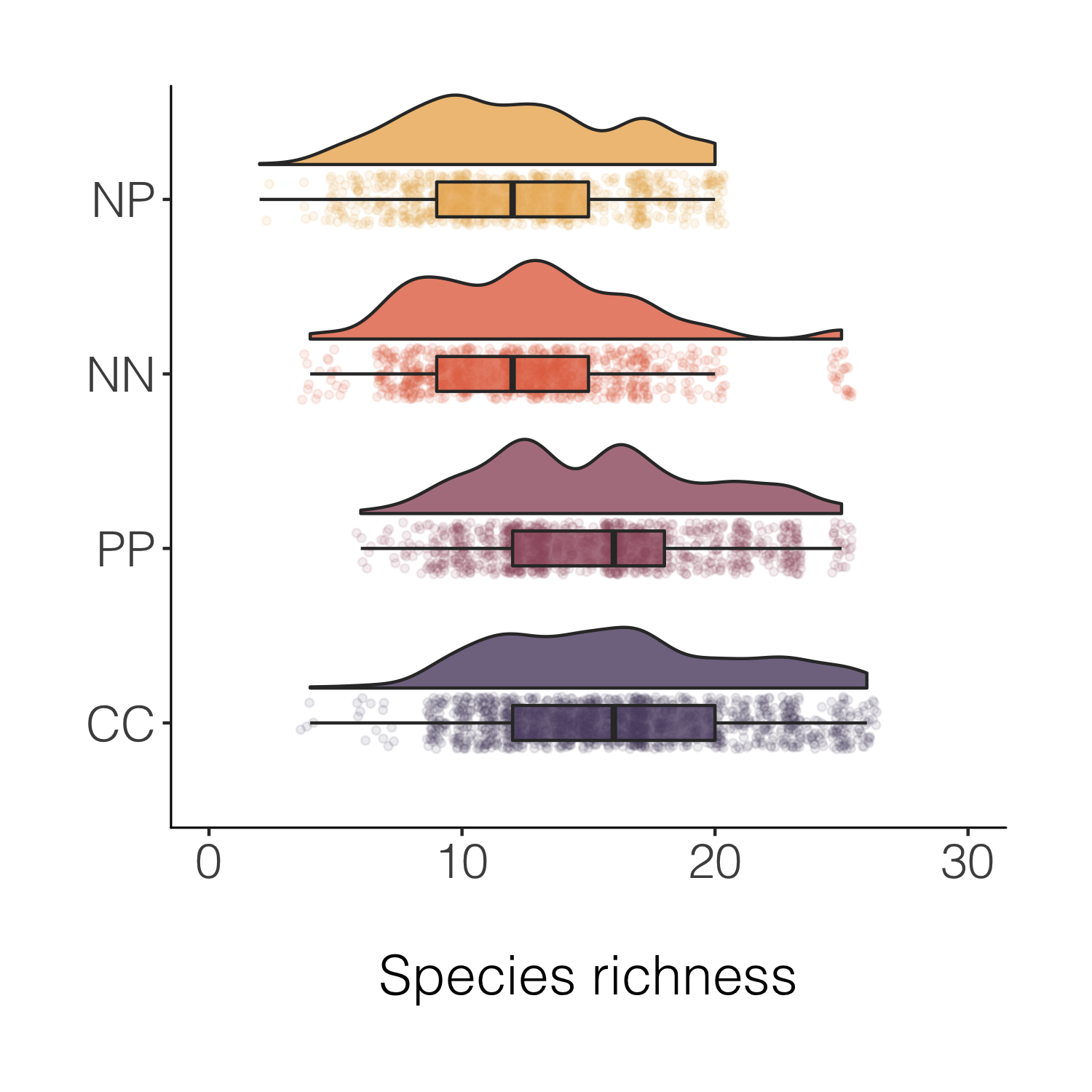Final stop along this specific beautification journey, for now at least! But before we move onto histograms, a note about another useful `tidyverse` feature - being able to quickly create a new variable based on conditions from more than one of the existing variables.

A data manipulation tip: Using `case_when()`, combined with `mutate`, is a great way to create new variables based on one or more conditions from other variables.

``````# Create new columns based on a combo of conditions using case_when()
# A fictional example
alpine_magic <- niwot_richness %>% mutate(fairy_dust = case_when(fert == "PP" & hits > 5 ~ "Blue fairy dust",
fert == "CC" & hits > 15 ~ "The ultimate fairy dust"))

(distributions_magic <-
ggplot(data = alpine_magic,
aes(x = reorder(fairy_dust, desc(richness)), y = richness, fill = fairy_dust)) +
geom_flat_violin(position = position_nudge(x = 0.2, y = 0), alpha = 0.8) +
geom_point(aes(y = richness, color = fairy_dust),
position = position_jitter(width = 0.15), size = 1, alpha = 0.1) +
geom_boxplot(width = 0.2, outlier.shape = NA, alpha = 0.8) +
labs(y = "\nSpecies richness", x = NULL) +
guides(fill = FALSE, color = FALSE) +
scale_y_continuous(limits = c(0, 30)) +
scale_fill_manual(values = c("turquoise4", "magenta4")) +
scale_colour_manual(values = c("turquoise4", "magenta4")) +
coord_flip() +
theme_niwot())
``````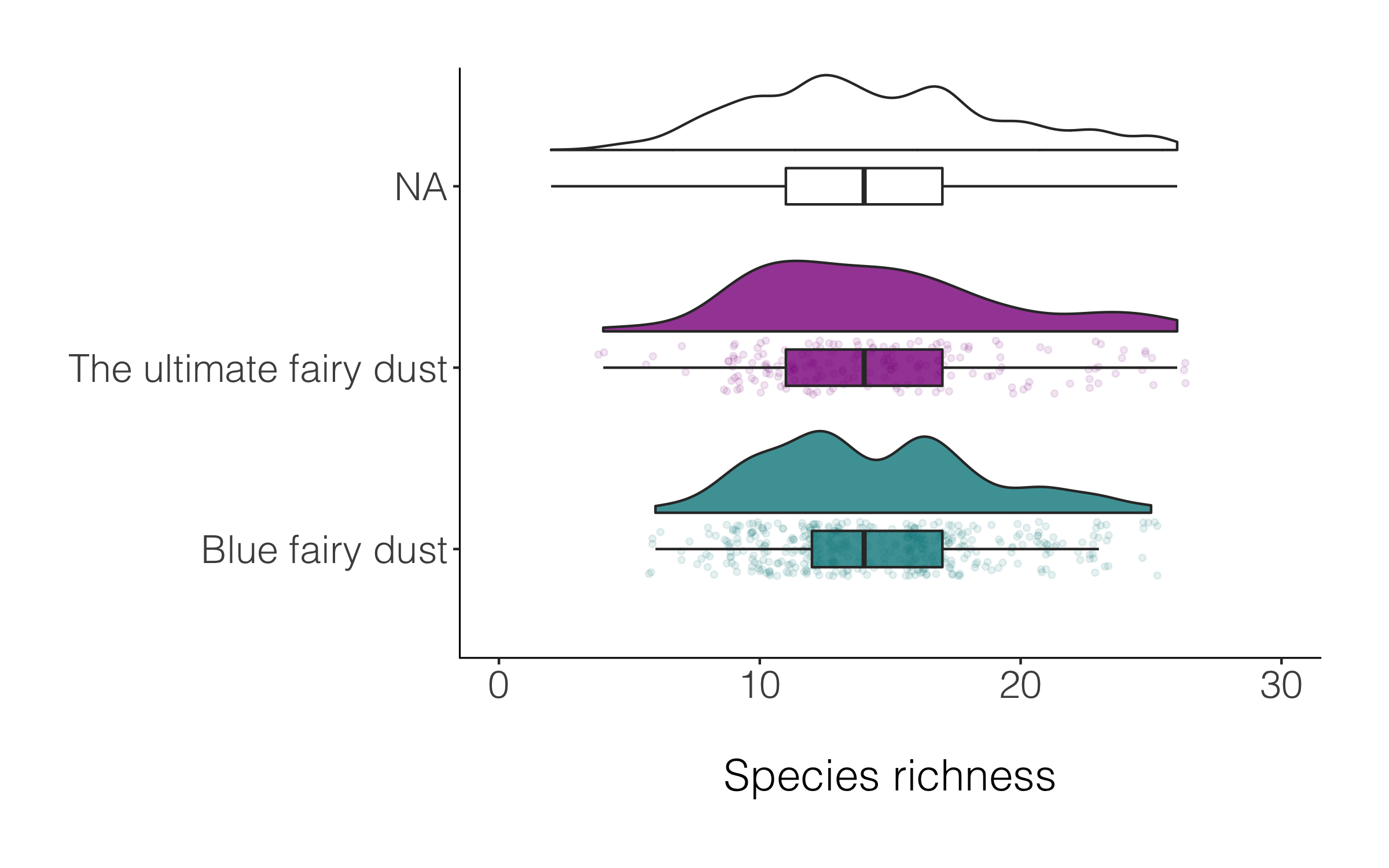A data manipulation tip: Often we have missing values, or not everything has a category, for example in the magic plot above, many of the species are classified as `NA`. If we want to drop those records, we can use `drop_na()` and in the brackets specify which specific column(s) should be the evaluator.

``````alpine_magic_only <- alpine_magic %>% drop_na(fairy_dust)

(distributions_magic2 <-
ggplot(data = alpine_magic_only,
aes(x = reorder(fairy_dust, desc(richness)), y = richness, fill = fairy_dust)) +
geom_flat_violin(position = position_nudge(x = 0.2, y = 0), alpha = 0.8) +
geom_point(aes(y = richness, color = fairy_dust),
position = position_jitter(width = 0.15), size = 1, alpha = 0.1) +
geom_boxplot(width = 0.2, outlier.shape = NA, alpha = 0.8) +
labs(y = "\nSpecies richness", x = NULL) +
guides(fill = FALSE, color = FALSE) +
scale_y_continuous(limits = c(0, 30)) +
scale_fill_manual(values = c("turquoise4", "magenta4")) +
scale_colour_manual(values = c("turquoise4", "magenta4")) +
coord_flip() +
theme_niwot())

ggsave(distributions_magic2, filename = "distributions_magic2.png",
height = 5, width = 5)
``````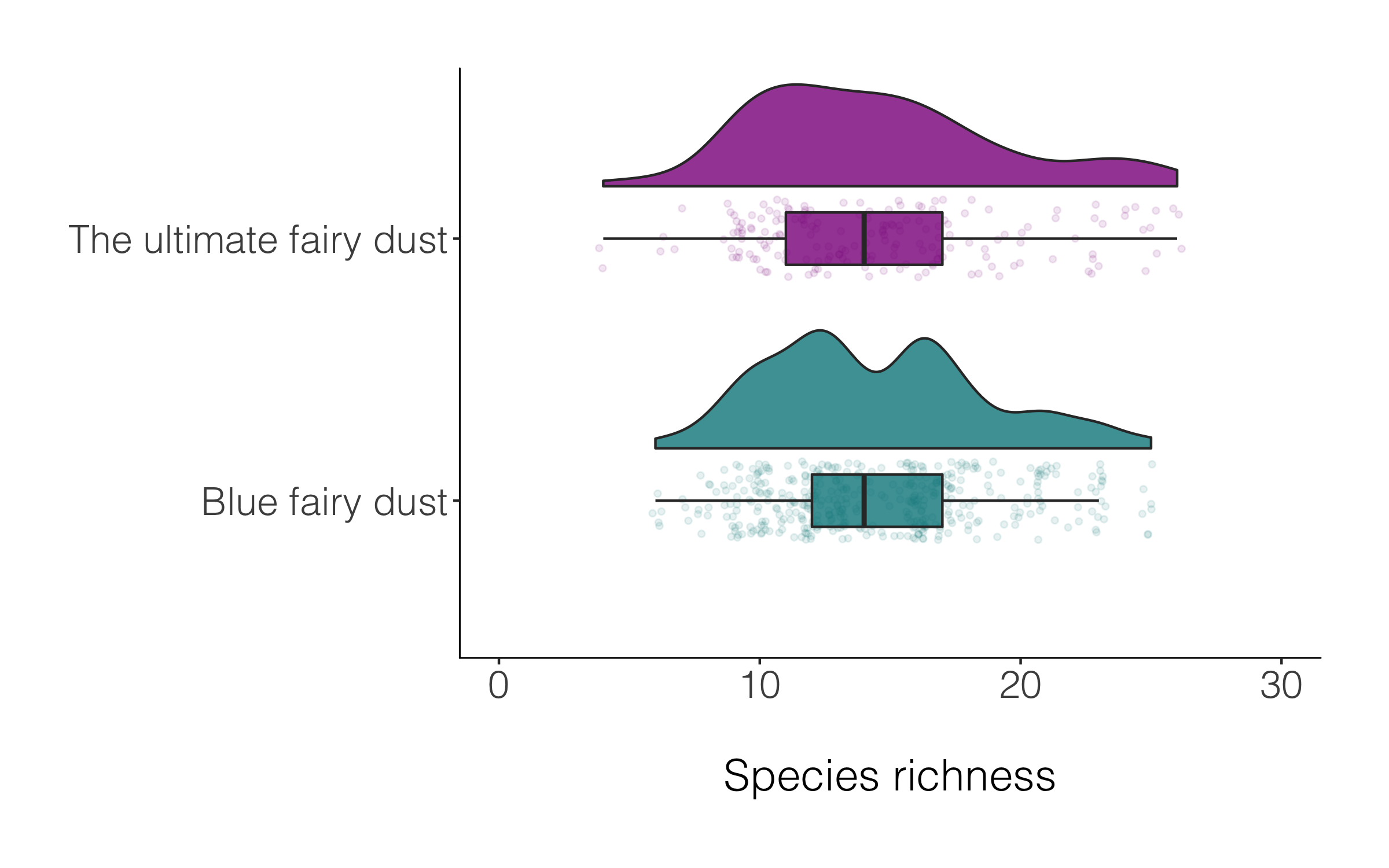Raining or not, both versions of the raincloud plot look alright, so like many things in data viz, a matter of personal preferenece.

## Make, customise and annotate histograms

A histogram is a simple but mighty plot and for the times when violins and rainclouds are a bit too busy, they can be an excellent way to communicate patterns in your data. Here’s the journey (one of the many possible journeys) of a histogram.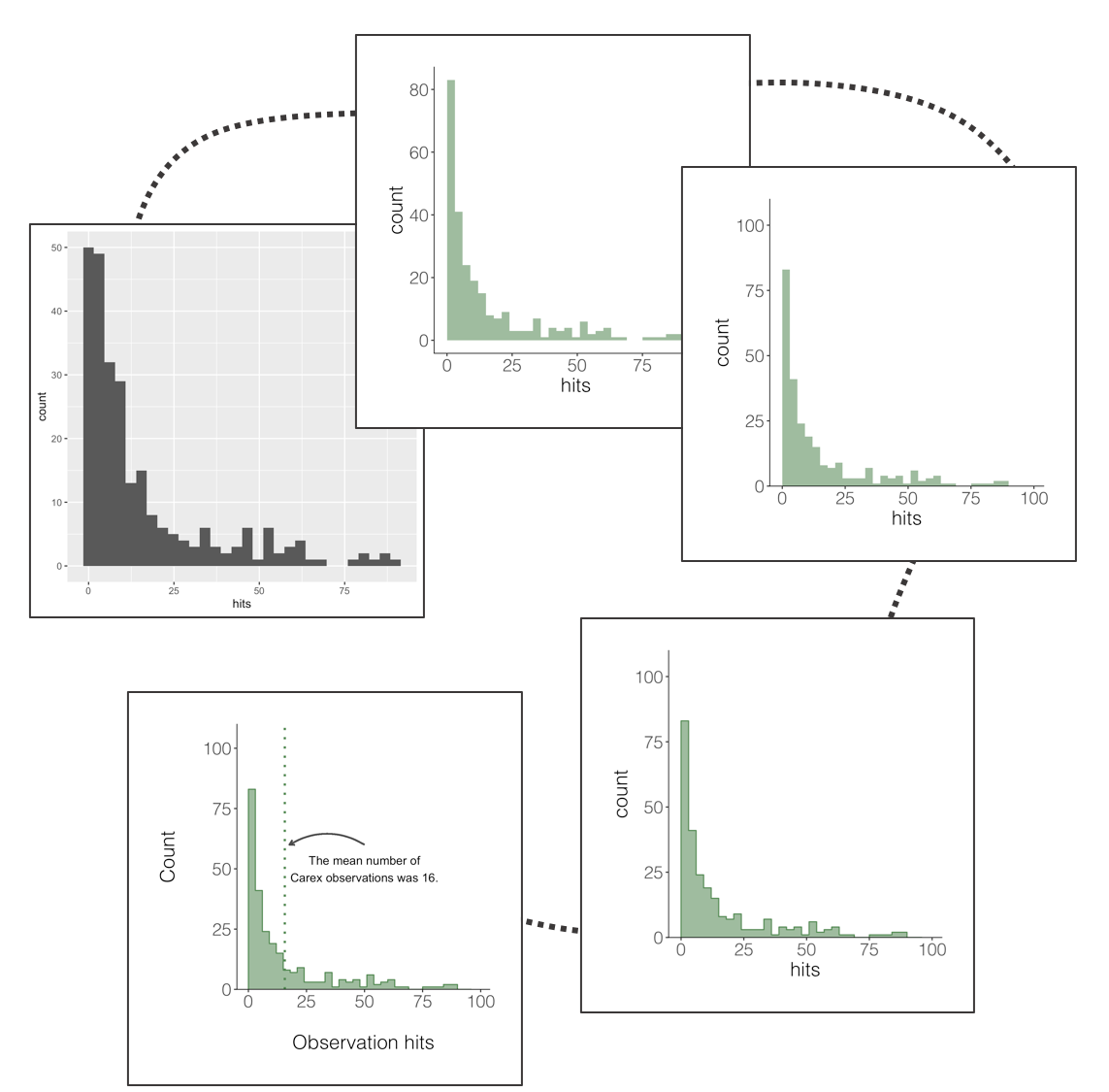A data manipulation tip: Whenever we go about doing our science, it’s important to be transparent and aware of our sample size and any limitations and strengths that come with it. A very useful function to count the number of observations (rows in your data frame) is `tally()`, which combined with `group_by()` creates a nice and quick summary of how many observations there are in the different categories in your data.

``````# Calculate number of data records per plot per year
# Using the tally() function

observations <- niwot_plant_exp %>% group_by(USDA_Scientific_Name) %>%
tally() %>% arrange(desc(n))  # rearanging the data frame so that the most common species are first
``````

A data manipulation tip: Filtering and selecting just certain parts of our data is a task we do often, and thanks to the `tidyverse`, there are efficient ways to filter based on a certain pattern. For example, let’s imagine we want just the records for plant species from the Carex family - we don’t really want to spell them all out, and we might miss some if we do. So we can just filter for anything that contains the word `Carex`.

``````# Filtering out just Carex species
carex <- niwot_plant_exp %>%
filter(str_detect(USDA_Scientific_Name, pattern = "Carex"))
``````

Now that we have a data frame with just Carex plant observations, we can visualise the distribution of how frequently these species are observed across the plots. In these data, that means plotting a histogram of the number of “hits” - how many times during the field data collection the pin used for observations “hit” a Carex species.

``````(histogram1 <- ggplot(carex, aes(x = hits)) +
geom_histogram())

ggsave(histogram1, filename = "histogram1.png",
height = 5, width = 5)
``````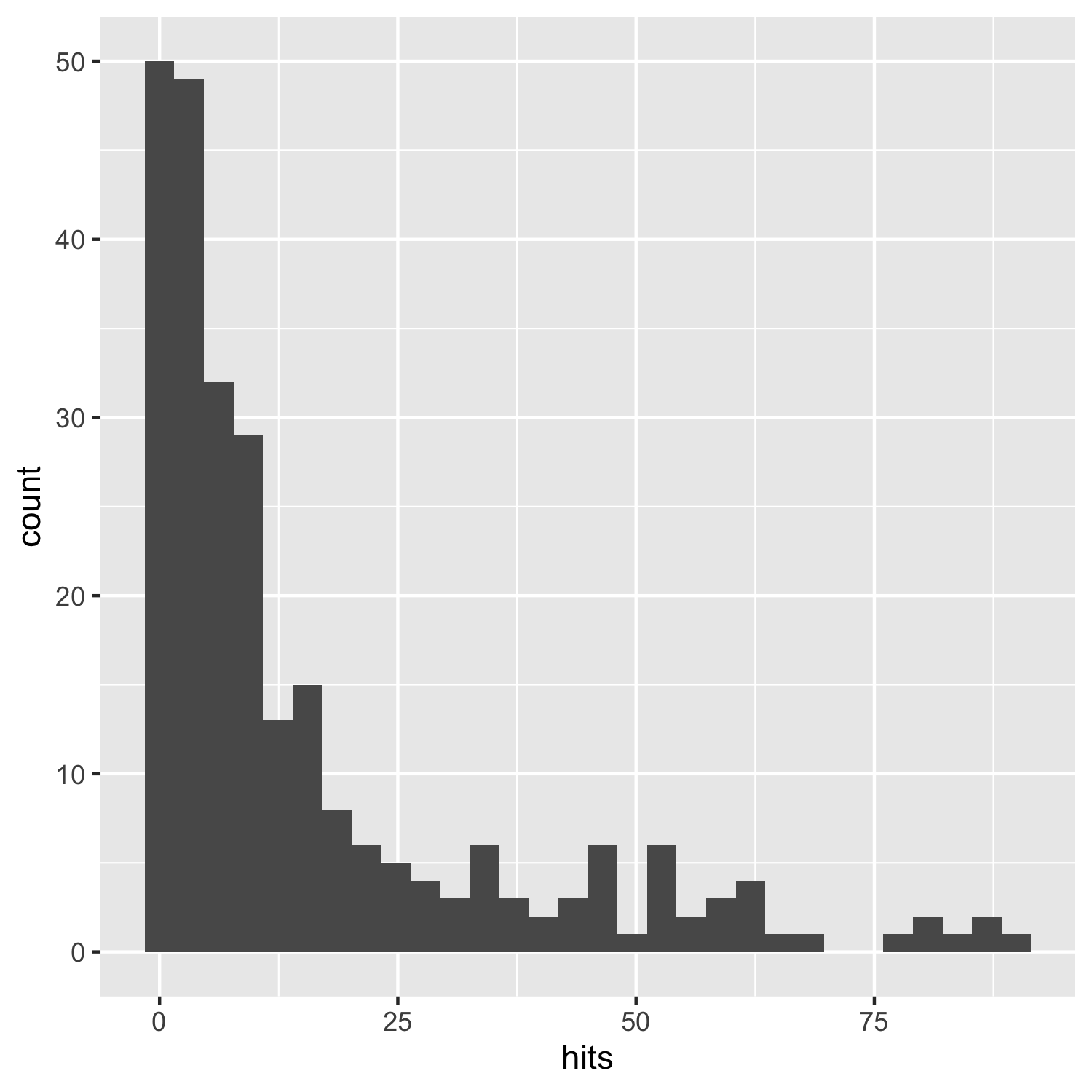This does the job, but it’s not particularly beautiful and everything is rather on the grey side.

With the growing popularity of `ggplot2`, one thing that stands out is that here we have used all of the default `ggplot2` options. Similarly, when we use the default `ggplot2` colours like in the violin plots earlier on, most people now recognise those, so you risk people immediately thinking “I know those colours, ggplot!” versus pausing to actually take in your scientific message. So making a graph as “yours” as possible can make your work more memorable!

``````(histogram2 <- ggplot(carex, aes(x = hits)) +
geom_histogram(alpha = 0.6,
breaks = seq(0, 100, by = 3),
# Choosing a Carex-like colour
fill = "palegreen4") +
theme_niwot())

ggsave(histogram2, filename = "histogram2.png",
height = 5, width = 5)
``````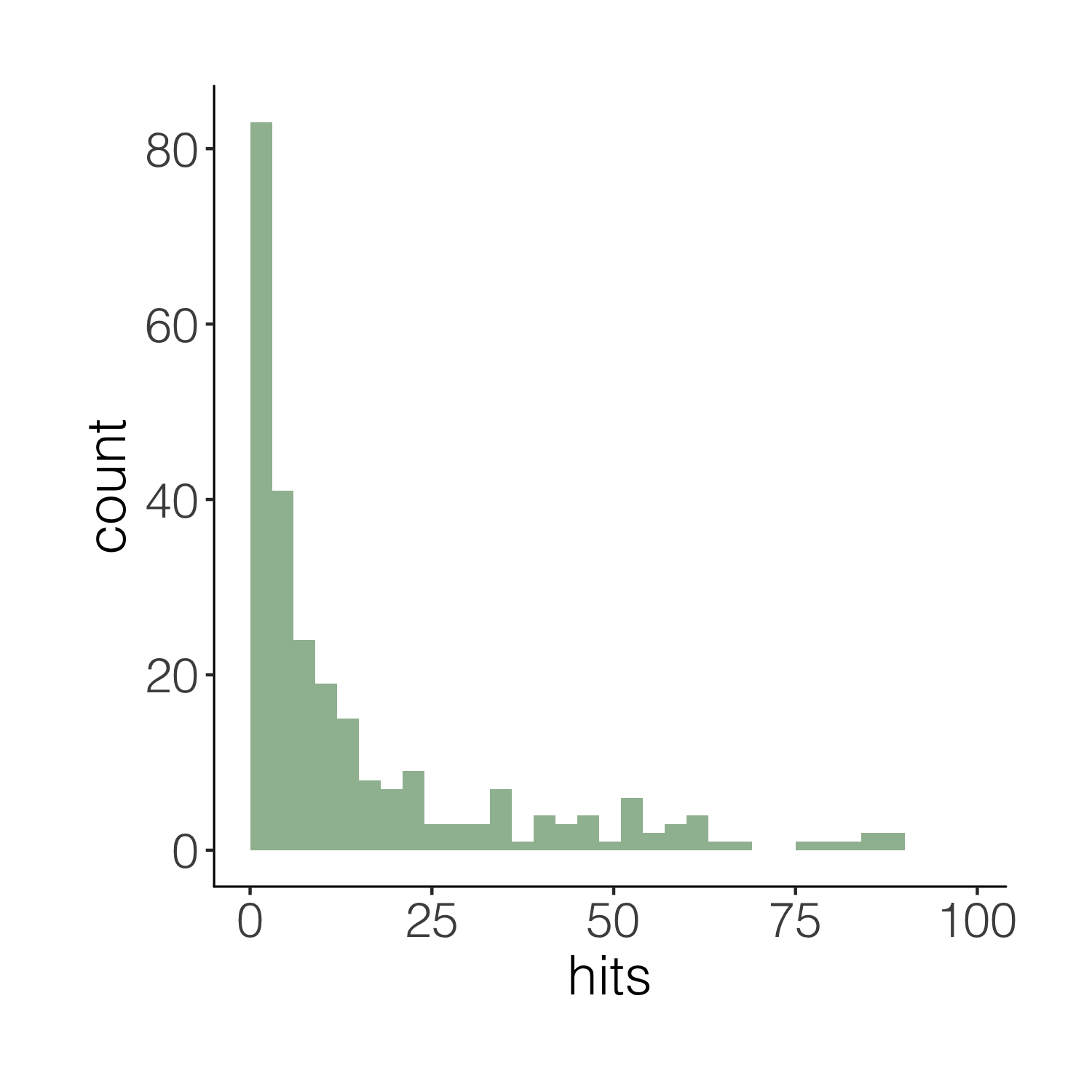This one is definitely nicer to look at, but our histogram is floating in space. We can easily remove the empty space.

``````(histogram3 <- ggplot(carex, aes(x = hits)) +
geom_histogram(alpha = 0.6,
breaks = seq(0, 100, by = 3),
fill = "palegreen4") +
theme_niwot() +
scale_y_continuous(limits = c(0, 100), expand = expand_scale(mult = c(0, 0.1))))
# the final line of code removes the empty blank space below the bars)

ggsave(histogram3, filename = "histogram3.png",
height = 5, width = 5)
``````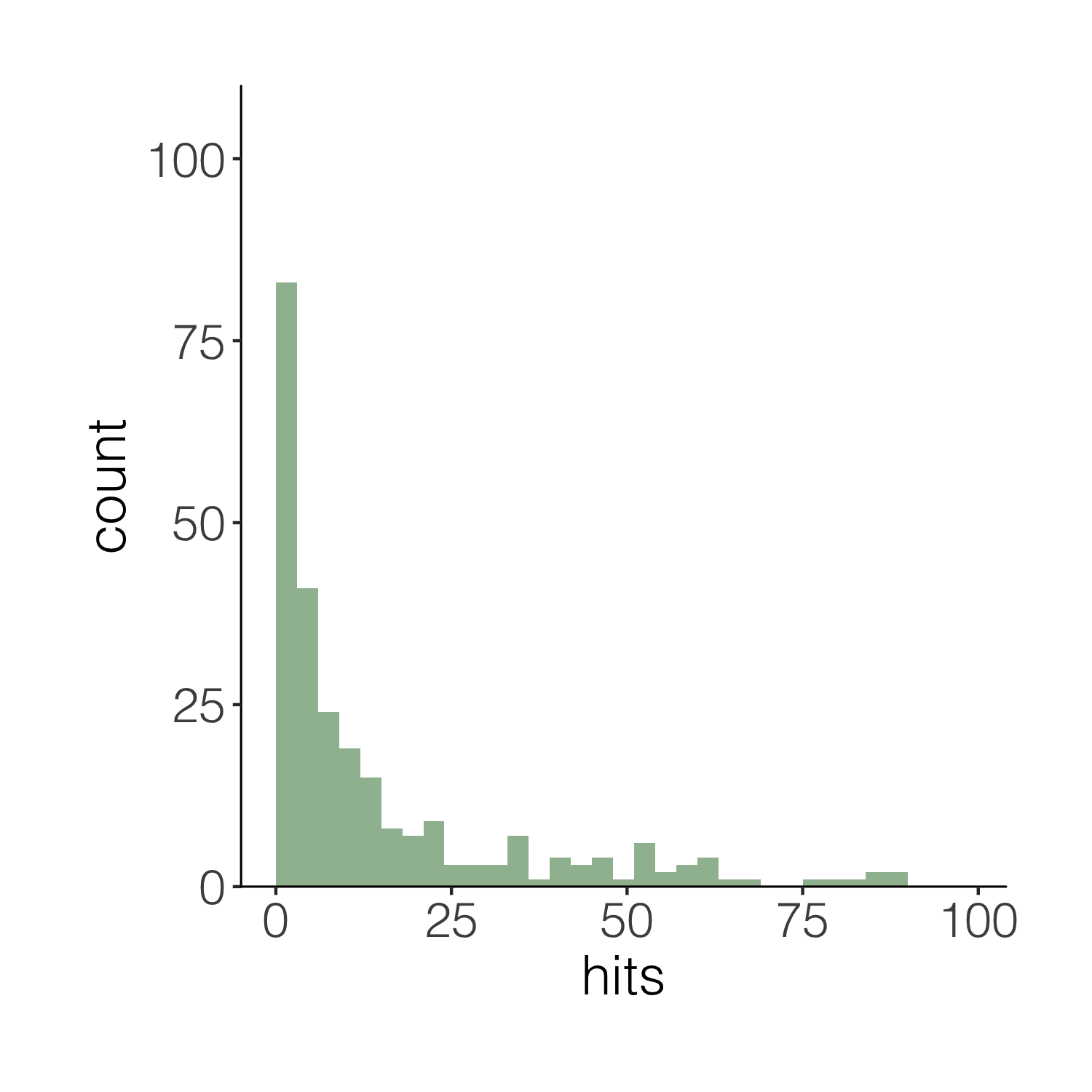Now imagine you want to have a darker green outline around the whole histogram - not around each individual bin, but the whole shape. It’s the little things that add up to make nice graphs! We can use `geom_step()` to create the histogram outline, but we have to put the steps in a data frame first. The three lines of code below are a bit of a cheat to create the histogram outline effect. Check out the object `d1` to see what we’ve made.

``````# Adding an outline around the whole histogram
h <- hist(carex\$hits, breaks = seq(0, 100, by = 3), plot = FALSE)
d1 <- data.frame(x = h\$breaks, y = c(h\$counts, NA))
d1 <- rbind(c(0, 0), d1)
``````

When we want to plot data from different data frames in the same graph, we have to move the data frame from the main `ggplot()` call to the specific part of the graph where we want to use each dataset. Compare the code below with the code for the previous versions of the histograms to spot the difference.

``````(histogram4 <- ggplot(carex, aes(x = hits)) +
geom_histogram(alpha = 0.6,
breaks = seq(0, 100, by = 3),
fill = "palegreen4") +
theme_niwot() +
scale_y_continuous(limits = c(0, 100), expand = expand_scale(mult = c(0, 0.1))) +
# Adding the outline
geom_step(data = d1, aes(x = x, y = y),
stat = "identity", colour = "palegreen4"))

summary(d1) # it's fine, you can ignore the warning message
# it's because some values don't have bars
# thus there are missing "steps" along the geom_step path

ggsave(histogram4, filename = "histogram4.png",
height = 5, width = 5)
``````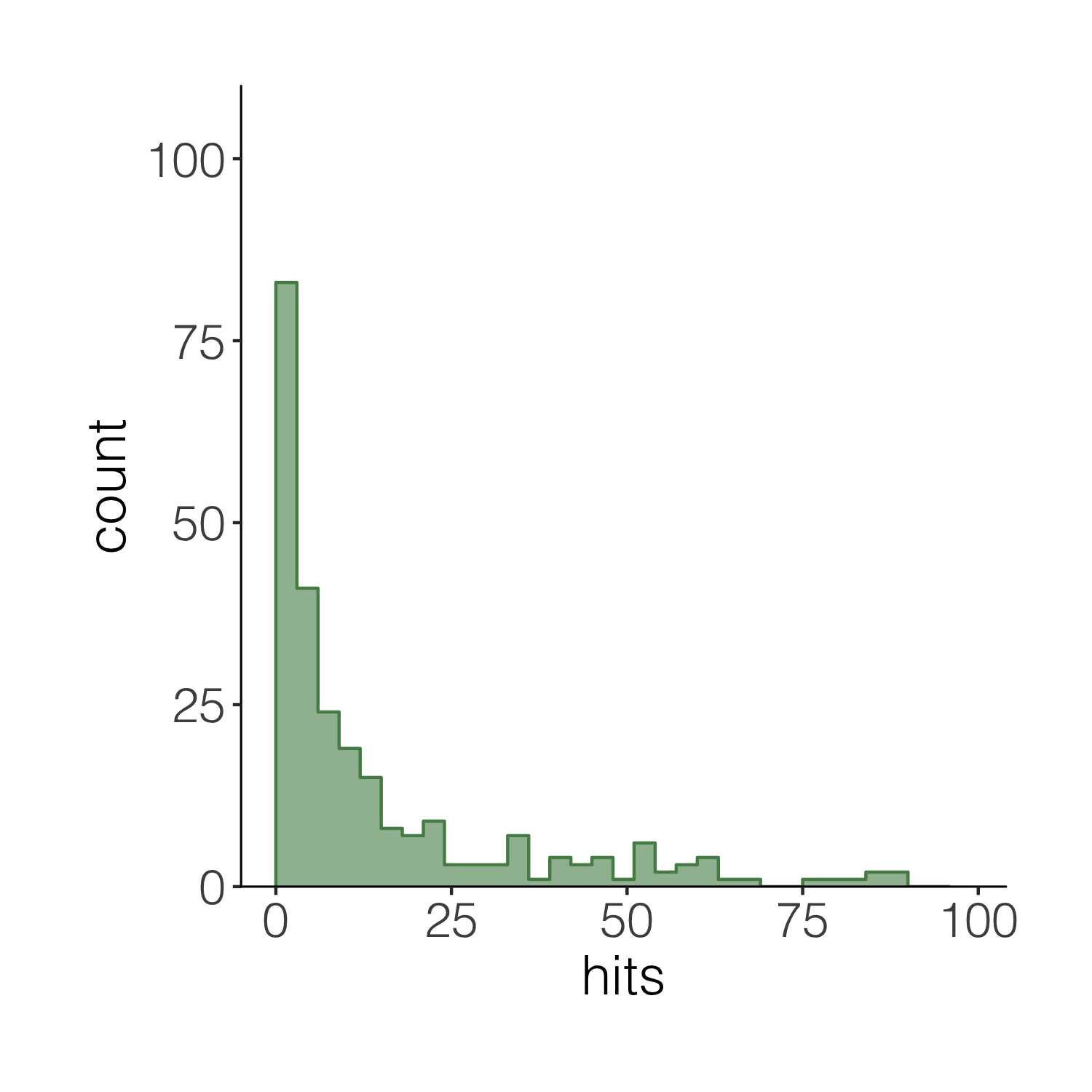We can also add a line for the mean number of hits and add an annotation on the graph so that people can quickly see what the line means.

``````(histogram5 <- ggplot(carex, aes(x = hits)) +
geom_histogram(alpha = 0.6,
breaks = seq(0, 100, by = 3),
fill = "palegreen4") +
theme_niwot() +
scale_y_continuous(limits = c(0, 100), expand = expand_scale(mult = c(0, 0.1))) +
geom_step(data = d1, aes(x = x, y = y),
stat = "identity", colour = "palegreen4") +
geom_vline(xintercept = mean(carex\$hits), linetype = "dotted",
colour = "palegreen4", size = 1) +
# Adding in a text allocation - the coordinates are based on the x and y axes
annotate("text", x = 50, y = 50, label = "The mean number of\nCarex observations was 16.") +
# "\n" creates a line break
geom_curve(aes(x = 50, y = 60, xend = mean(carex\$hits) + 2, yend = 60),
arrow = arrow(length = unit(0.07, "inch")), size = 0.7,
color = "grey30", curvature = 0.3) +
labs(x = "\nObservation hits", y = "Count\n"))
# Similarly to the annotation, the curved line follows the plot's coordinates
# Have a go at changing the curve parameters to see what happens

ggsave(histogram5, filename = "histogram5.png",
height = 5, width = 5)
``````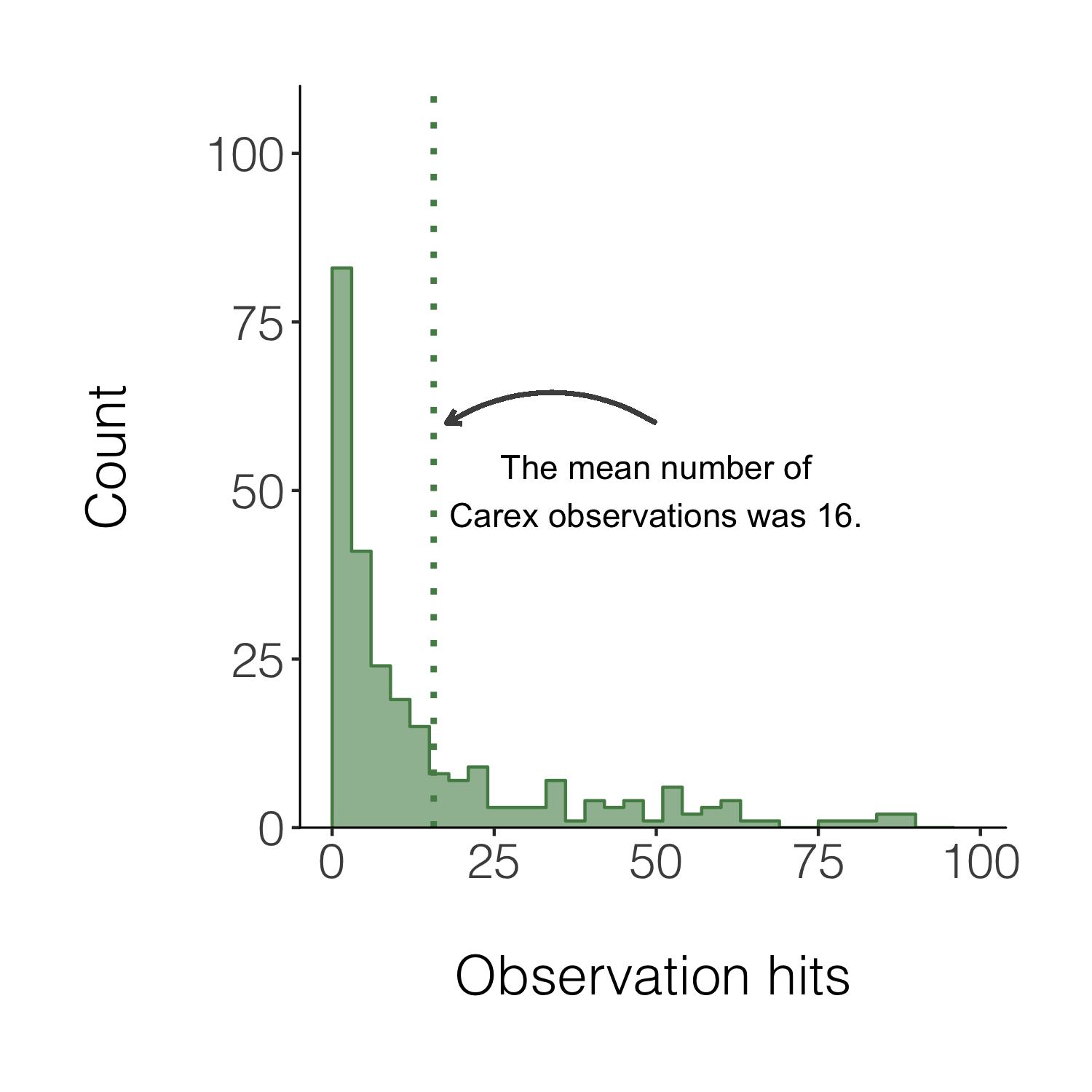## 4. Format and manipulate large datasets

Next up, we will focus on how to efficiently format, manipulate and visualise large datasets. We will use the `tidyr` and `dplyr` packages to clean up data frames and calculate new variables. We will use the `broom` and `purr` packages to make the modelling of thousands of population trends more efficient.

We will be working with bird population data (abundance over time) from the Living Planet Database, bird trait data from the Elton Database, and emu occurrence data from the Global Biodiversity Information Facility, all of which are publicly available datasets.

First, we will format the bird population data, calculate a few summary variables and explore which countries have the most population time-series and what is their average duration.

Here are the packages we need. Note that not all `tidyverse` packages load automatically with `library(tidyverse)` - only the core ones do, so you need to load `broom` separately. If you don’t have some of the packages installed, you can install them using `ìnstall.packages("package-name")`. One of the packages is only available on `GitHub`, so you can use `install_github()` to install it. In general, if you ever have troubles installing packages from CRAN (that’s where packages come from by default when using `install.packages()`), you can try googling the package name and “github” and installing it from its `GitHub` repo, sometimes that works!

#### Load population trend data

Now we’re ready to load in the rest of the data needed for this tutorial!

``````bird_pops <- read.csv("bird_pops.csv")
``````

We can check out what the data look like now, either by clicking on the objects name on the right in the list in your working environment, or by running `View(bird_pops)` in the console.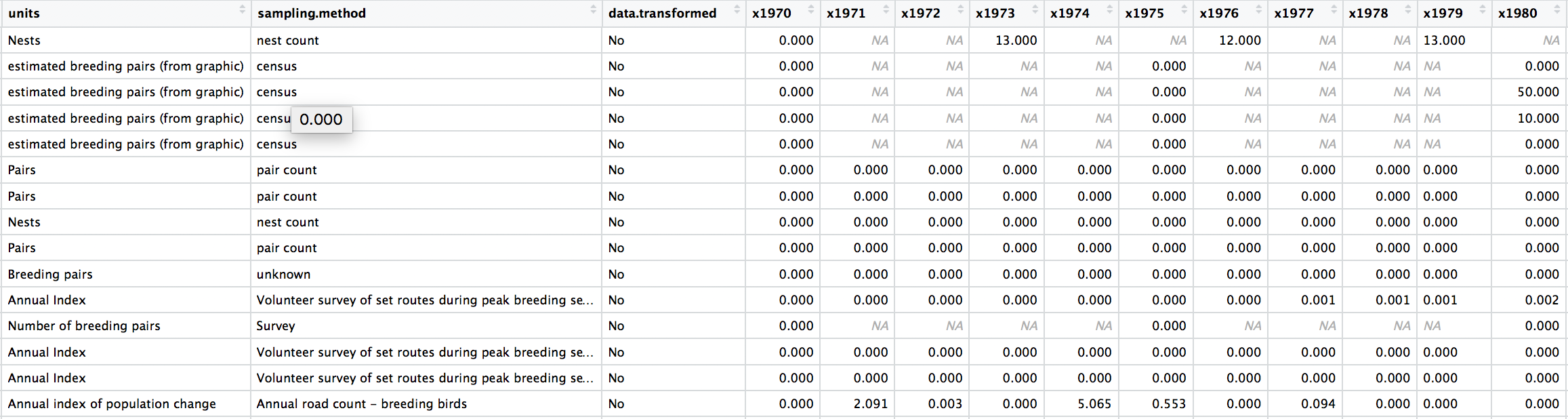The data are in a wide format (each row contains a population that has been monitored over time and towards the right of the data frame there are lots of columns with population estimates for each year) and the column names are capitalised. Whenever working with data from different sources, chances are each dataset will follow a different column naming system, which can get confusing later on, so in general it is best to pick whatever naming system works for you and apply that to all datasets before you start working with them.

``````# Data formatting ----
# Rename variable names for consistency
names(bird_pops)
names(bird_pops) <- tolower(names(bird_pops))
names(bird_pops)
``````

To make these data “tidy” (one column per variable and not the current wide format), we can use `gather()` to transform the data so there is a new column containing all the years for each population and an adjacent column containing all the population estimates for those years.

This takes our original dataset `bird_pops` and creates a new column called `year`, fills it with column names from columns `26:70` and then uses the data from these columns to make another column called `pop`.

``````bird_pops_long <- gather(data = bird_pops, key = "year", value = "pop", 27:71)

# Examine the tidy data frame
``````

Because column names are coded in as characters, when we turned the column names (`1970`, `1971`, `1972`, etc.) into rows, R automatically put an `X` in front of the numbers to force them to remain characters. We don’t want that, so to turn `year` into a numeric variable, use the `parse_number()` function from the `readr` package.

``````# Get rid of the X in front of years
# *** parse_number() from the readr package in the tidyverse ***
bird_pops_long\$year <- parse_number(bird_pops_long\$year)
``````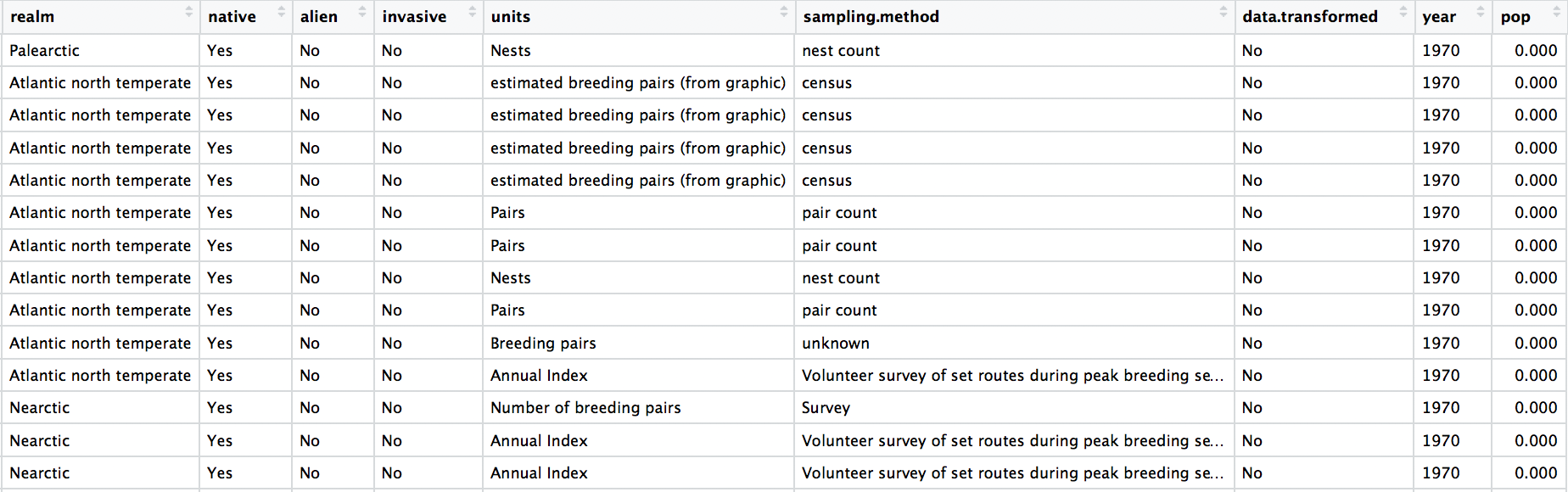Check out the data frame again to make sure the years really look like years. As you’re looking through, you might notice something else. We have many columns in the data frame, but there isn’t a column with the species’ name. We can make one super quickly, since there are already columns for the genus and the species.

``````# Create new column with genus and species together
bird_pops_long\$species.name <- paste(bird_pops_long\$genus, bird_pops_long\$species, sep = " ")
``````

We can tidy up the data a bit more and create a few new columns with useful information. Whenever we are working with datasets that combine multiple studies, it’s useful to know when they each started, what their duration was, etc. Here we’ve combined all of that into one “pipe” (lines of code that use the piping operator `%>%`). The pipes always take whatever has come out of the previous pipe (or the first object you’ve given the pipe), and at the end of all the piping, out comes a tidy data frame with useful information.

``````# *** piping from from dplyr
bird_pops_long <- bird_pops_long %>%
# Remove duplicate rows
# *** distinct() function from dplyr
distinct() %>%
# remove NAs in the population column
# *** filter() function from dplyr
filter(is.finite(pop)) %>%
# Group rows so that each group is one population
# *** group_by() function from dplyr
group_by(id) %>%
# Make some calculations
# *** mutate() function from dplyr
mutate(maxyear = max(year), minyear = min(year),
# Calculate duration
duration = maxyear - minyear,
# Scale population trend data
scalepop = (pop - min(pop))/(max(pop) - min(pop))) %>%
# Keep populations with >5 years worth of data and calculate length of monitoring
filter(is.finite(scalepop),
length(unique(year)) > 5) %>%
# Remove any groupings you've greated in the pipe
ungroup()

``````

Now we can calculate some finer-scale summary statistics. Though we have the most ecological data we’ve ever had, there are still many remaining data gaps, and a lot of what we know about biodiversity is based on information coming from a small set of countries. Let’s check out which!

``````# Which countries have the most data
# Using "group_by()" to calculate a "tally"
# for the number of records per country
country_sum <- bird_pops %>% group_by(country.list) %>%
tally() %>%
arrange(desc(n))

country_sum[1:15,] # the top 15
``````

As we probably all expected, a lot of the data come from Western European and North American countries. Sometimes as we navigate our research questions, we go back and forth between combining (adding in more data) and extracting (filtering to include only what we’re interested in), so to mimic that, this tutorial will similarly take you on a combining and extracting journey, this time through Australia.

To get just the Australian data, we can use the `filter()` function. To be on the safe side, we can also combine it with `str_detect()`. The difference is that filter on its own will extract any rows with “Australia”, but it will miss rows that have e.g. “Australia / New Zealand” - occasions when the population study included multiple countries. In this case though, both ways of filtering return the same number of rows, but always good to check.

``````# Data extraction ----
aus_pops <- bird_pops_long %>%
filter(country.list == "Australia")

# Giving the object a new name so that you can compare
# and see that in this case they are the same
aus_pops2 <- bird_pops_long %>%
filter(str_detect(country.list, pattern = "Australia"))
``````

We are now ready to model how each population has changed over time. There are 4331 populations, so with this one code chunk, we will run 4331 models and tidy up their outputs. You can read through the line-by-line comments to get a feel for what each line of code is doing.

One specific thing to note is that when you add the `lm()` function in a pipe, you have to add `data = .`, which means use the outcome of the previous step in the pipe for the model.

``````# Calculate population change for each forest population
# 4331 models in one go!
# Using a pipe
aus_models <- aus_pops %>%
# Group by the key variables that we want to iterate over
# note that if we only include e.g. id (the population id), then we only get the
# id column in the model summary, not e.g. duration, latitude, class...
group_by(decimal.latitude, decimal.longitude, class,
species.name, id, duration, minyear, maxyear,
system, common.name) %>%
# Create a linear model for each group
# Extract model coefficients using tidy() from the
# *** tidy() function from the broom package ***
do(broom::tidy(lm(scalepop ~ year, .))) %>%
# Filter out slopes and remove intercept values
filter(term == "year") %>%
# Get rid of the column term as we don't need it any more
#  *** select() function from dplyr in the tidyverse ***
dplyr::select(-term) %>%
# Remove any groupings you've greated in the pipe
ungroup()

# Check out the model data frame
``````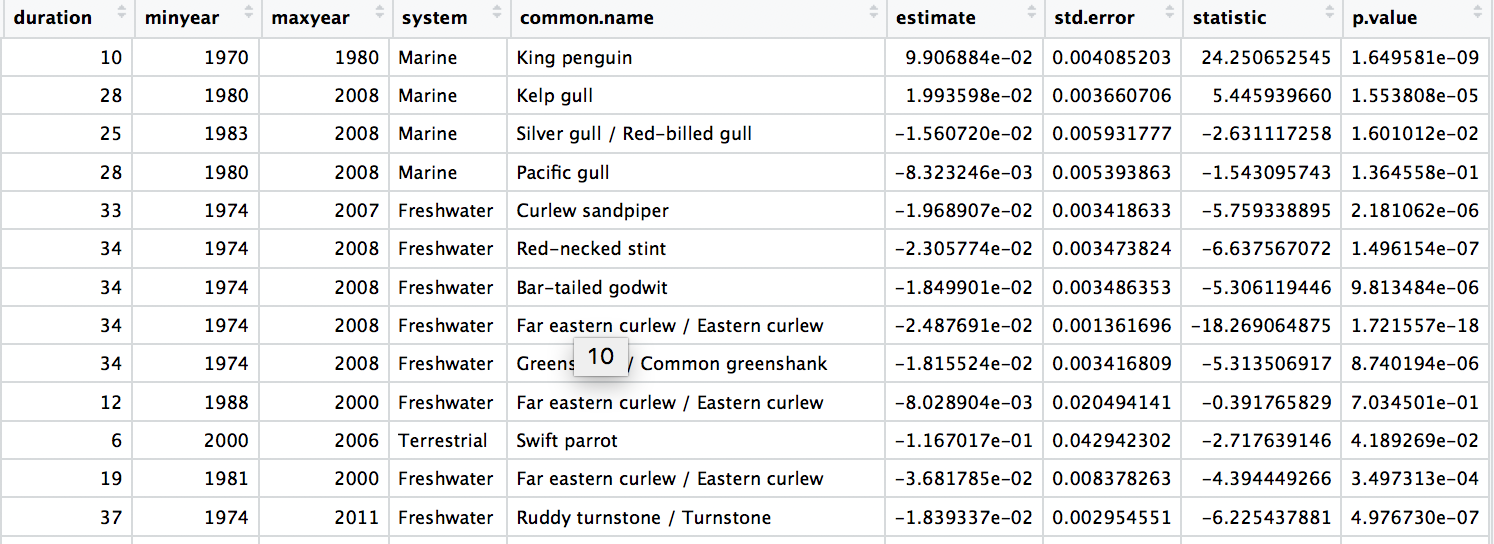## 5. Synthesise information from different databases

Answering research questions often requires combining data from different sources. For example, we’ve explored how bird abundance has changed over time across the monitored populations in Australia, but we don’t know whether certain groups of species might be more likely to increase or decrease. To find out, we can integrate the population trend data with information on species traits, in this case species’ diet preferences.

The various joining functions from the `dplyr` package are really useful for combining data. We will use `left_join` in this tutorial, but you can find out about all the other options by running ?join() and reading the help file. To join two datasets in a meaningful way, you usually need to have one common column in both data frames and then you join “by” that column.

``````# Data synthesis - traits! ----

# Tidying up the trait data
# similar to how we did it for the population data
colnames(bird_traits)
bird_traits <- bird_traits %>% rename(species.name = Scientific)
# rename is a useful way to change column names
# it goes new name =  old name
colnames(bird_traits)

# Select just the species and their diet
bird_diet <- bird_traits %>% dplyr::select(species.name, `Diet.5Cat`) %>%
distinct() %>% rename(diet = `Diet.5Cat`)

# Combine the two datasets
# The second data frame will be added to the first one
# based on the species column
bird_models_traits <- left_join(aus_models, bird_diet, by = "species.name") %>%
drop_na()
``````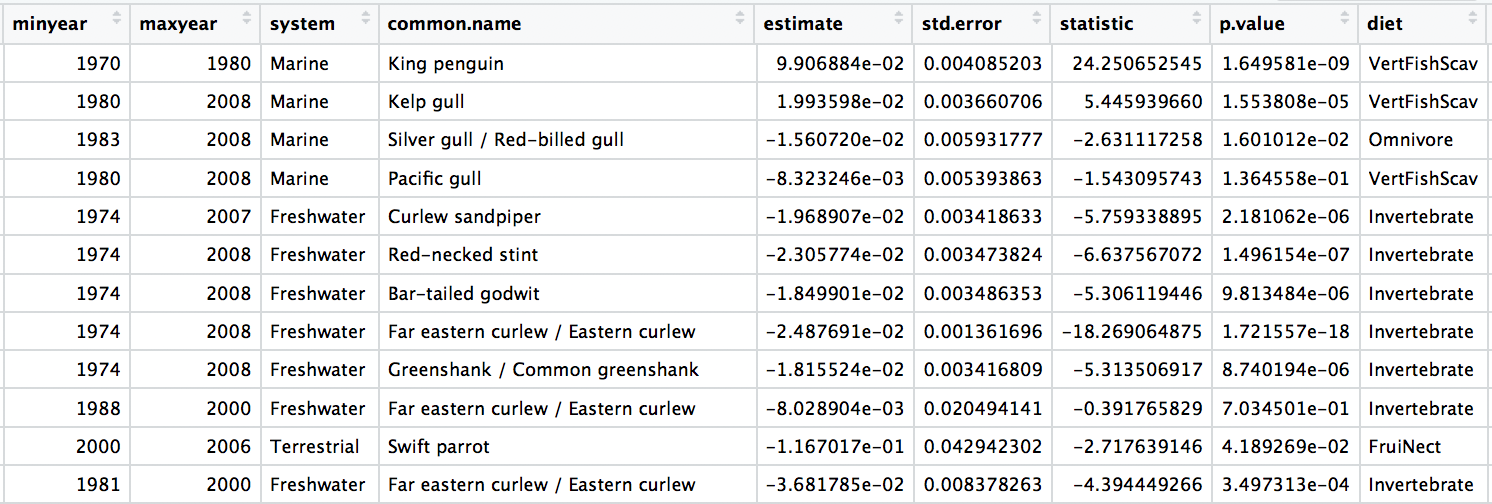Now we can explore how bird population trends vary across different feeding strategies. The graphs below are all different ways to answer the same question. Have a ponder about which graph you like the most.

``````(trends_diet <- ggplot(bird_models_traits, aes(x = diet, y = estimate,
colour = diet)) +
geom_boxplot())

(trends_diet <- ggplot(data = bird_models_traits, aes(x = diet, y = estimate,
colour = diet)) +
geom_jitter(size = 3, alpha = 0.3, width = 0.2))

``````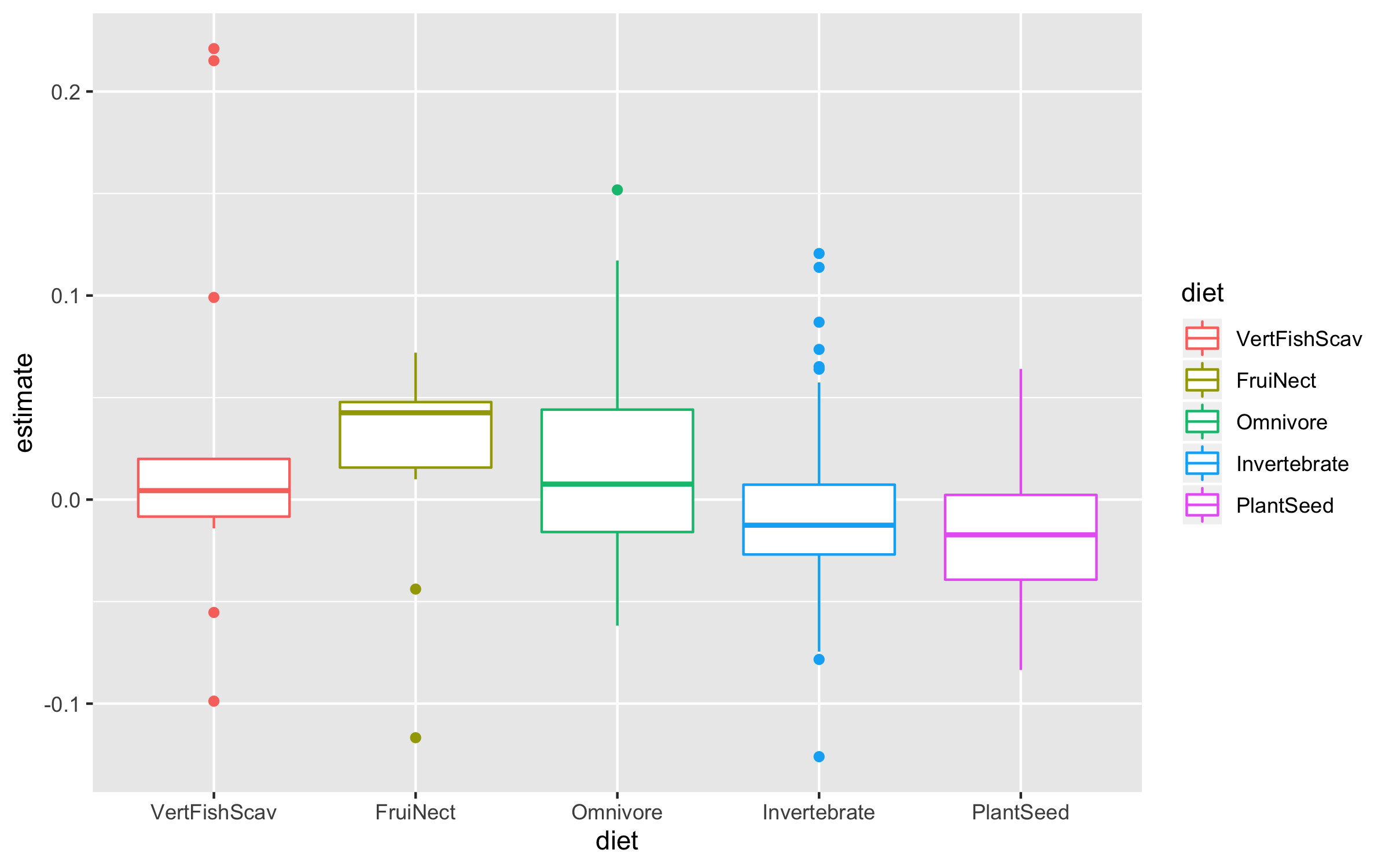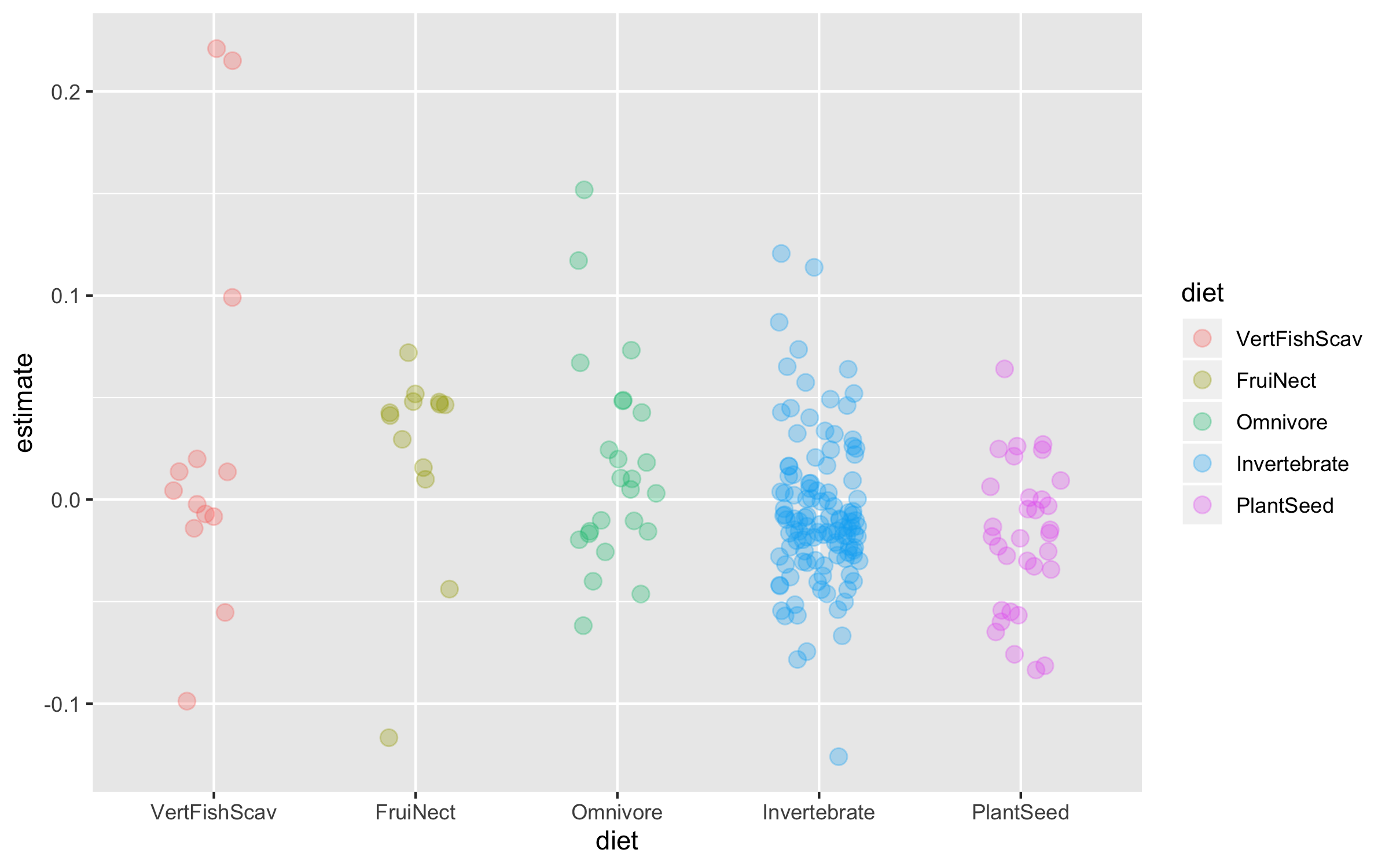To make the graph more informative, we can add a line for the overall mean population trend, and then we can easily compare how the diet-specific trends compare to the overall mean trend. We can also plot the mean trend per diet category and we can sort the graph so that it goes from declines to increases.

``````# Sorting the whole data frame by the mean trends
bird_models_traits <- bird_models_traits %>%
group_by(diet) %>%
mutate(mean_trend = mean(estimate)) %>%
ungroup() %>%
mutate(diet = fct_reorder(diet, -mean_trend))

# Calculating mean trends per diet categories
diet_means <- bird_models_traits %>% group_by(diet) %>%
summarise(mean_trend = mean(estimate)) %>%
arrange(mean_trend)
``````

Finally, we can also use `geom_segment` to connect the points for the mean trends to the line for the overall mean, so we can judge how far off each category is from the mean.

``````(trends_diet <- ggplot() +
geom_jitter(data = bird_models_traits, aes(x = diet, y = estimate,
colour = diet),
size = 3, alpha = 0.3, width = 0.2) +
geom_segment(data = diet_means,aes(x = diet, xend = diet,
y = mean(bird_models_traits\$estimate),
yend = mean_trend),
size = 0.8) +
geom_point(data = diet_means, aes(x = diet, y = mean_trend,
fill = diet), size = 5,
colour = "grey30", shape = 21) +
geom_hline(yintercept = mean(bird_models_traits\$estimate),
size = 0.8, colour = "grey30") +
geom_hline(yintercept = 0, linetype = "dotted", colour = "grey30") +
coord_flip() +
theme_clean() +
scale_colour_manual(values = wes_palette("Cavalcanti1")) +
scale_fill_manual(values = wes_palette("Cavalcanti1")) +
scale_y_continuous(limits = c(-0.23, 0.23),
breaks = c(-0.2, -0.1, 0, 0.1, 0.2),
labels = c("-0.2", "-0.1", "0", "0.1", "0.2")) +
scale_x_discrete(labels = c("Carnivore", "Fruigivore", "Omnivore", "Insectivore", "Herbivore")) +
labs(x = NULL, y = "\nPopulation trend") +
guides(colour = FALSE, fill = FALSE))
``````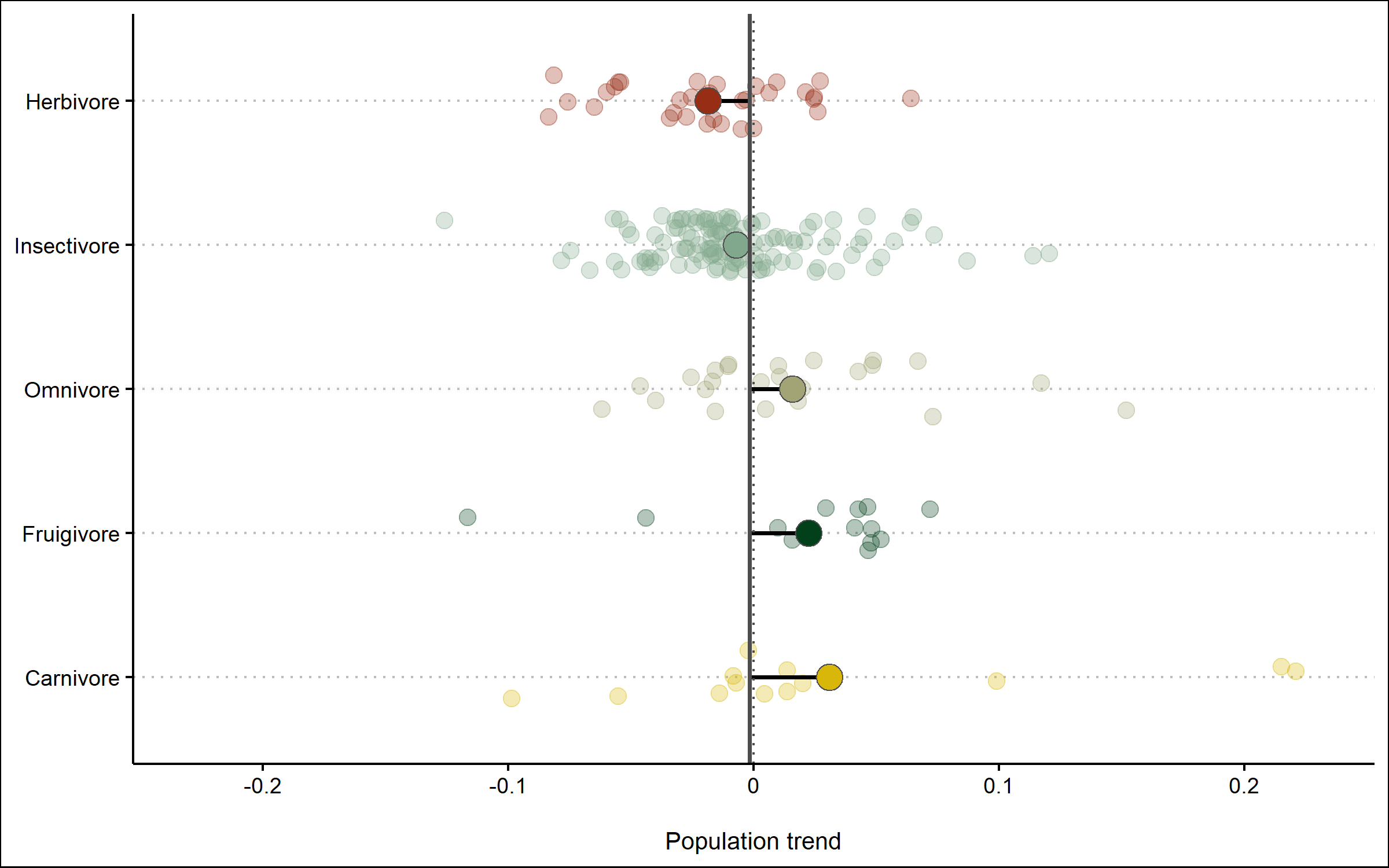Like before, we can save the graph using `ggsave`.

``````ggsave(trends_diet, filename = "trends_diet.png",
height = 5, width = 8)
``````

Knowing the sample size for each diet category is another useful bit of information, especially to support the spirit of open and transparent science. We can use `group_by()` and `tally()` to get the sample size numbers.

``````diet_sum <- bird_models_traits %>% group_by(diet) %>%
tally()
``````

Now that we know the numbers, we can visualise them. A barplot would be a classic way to do that, the second option present here - the area graph - is another option. Both can work well depending on the specific occasion, but the area graph does a good job at quickly communicating which categories are overrepresented and which - underrepresented.

``````(diet_bar <- ggplot(diet_sum, aes(x = diet, y = n,
colour = diet,
fill = diet)) +
geom_bar(stat = "identity") +
scale_colour_manual(values = wes_palette("Cavalcanti1")) +
scale_fill_manual(values = wes_palette("Cavalcanti1")) +
guides(colour = FALSE))

(diet_area <- ggplot(diet_sum, aes(area = n, fill = diet, label = n,
subgroup = diet)) +
geom_treemap() +
geom_treemap_subgroup_border(colour = "white", size = 1) +
geom_treemap_text(colour = "white", place = "center", reflow = T) +
scale_colour_manual(values = wes_palette("Cavalcanti1")) +
scale_fill_manual(values = wes_palette("Cavalcanti1")) +
guides(fill = FALSE))  # this removes the colour legend
# later on we will combine multiple plots so there is no need for the legend
# to be in twice

# To display the legend, just remove the guides() line:
(diet_area <- ggplot(diet_sum, aes(area = n, fill = diet, label = n,
subgroup = diet)) +
geom_treemap() +
geom_treemap_subgroup_border(colour = "white", size = 1) +
geom_treemap_text(colour = "white", place = "center", reflow = T) +
scale_colour_manual(values = wes_palette("Cavalcanti1")) +
scale_fill_manual(values = wes_palette("Cavalcanti1")))

ggsave(diet_area, filename = "diet_area.png",
height = 5, width = 8)
``````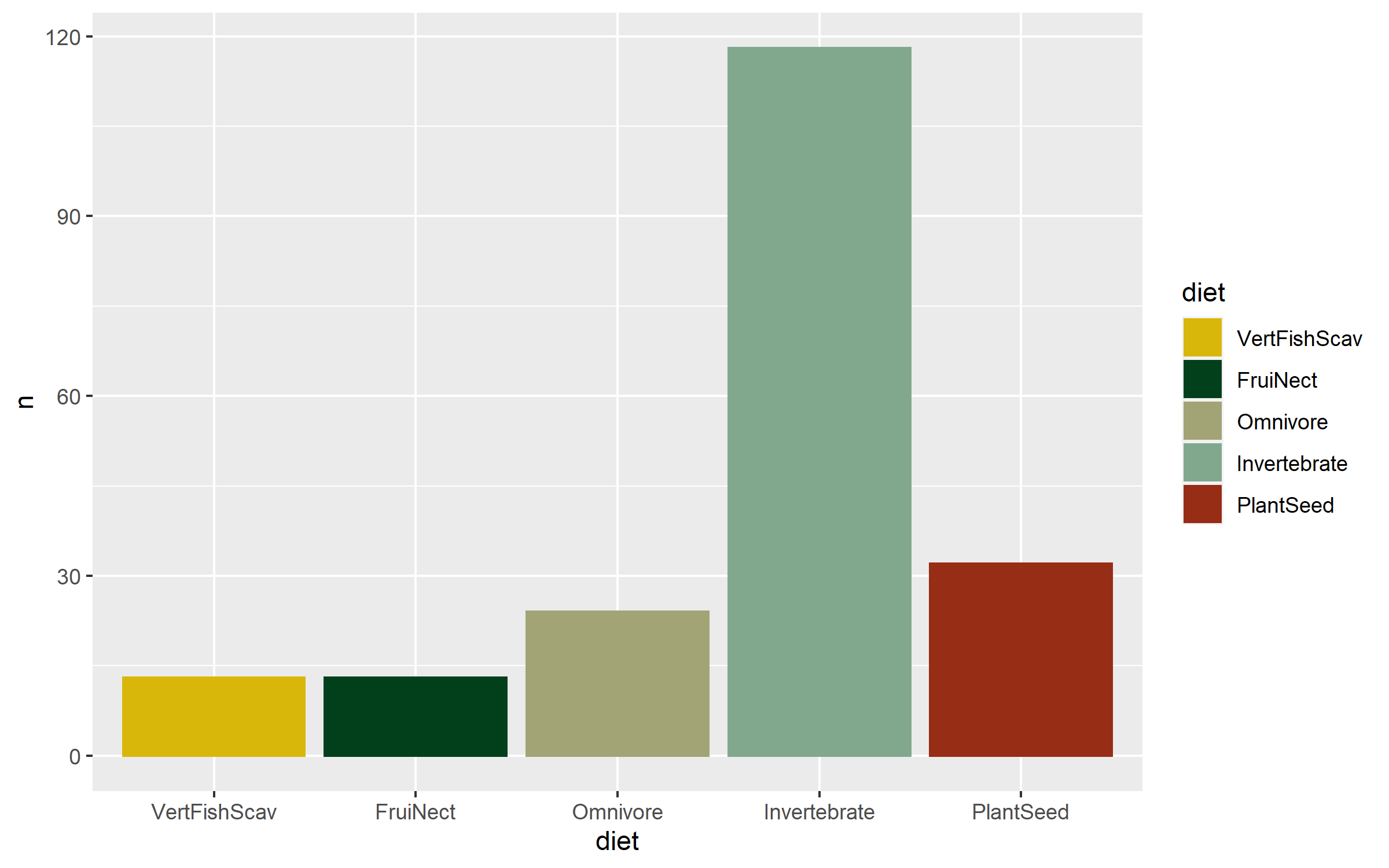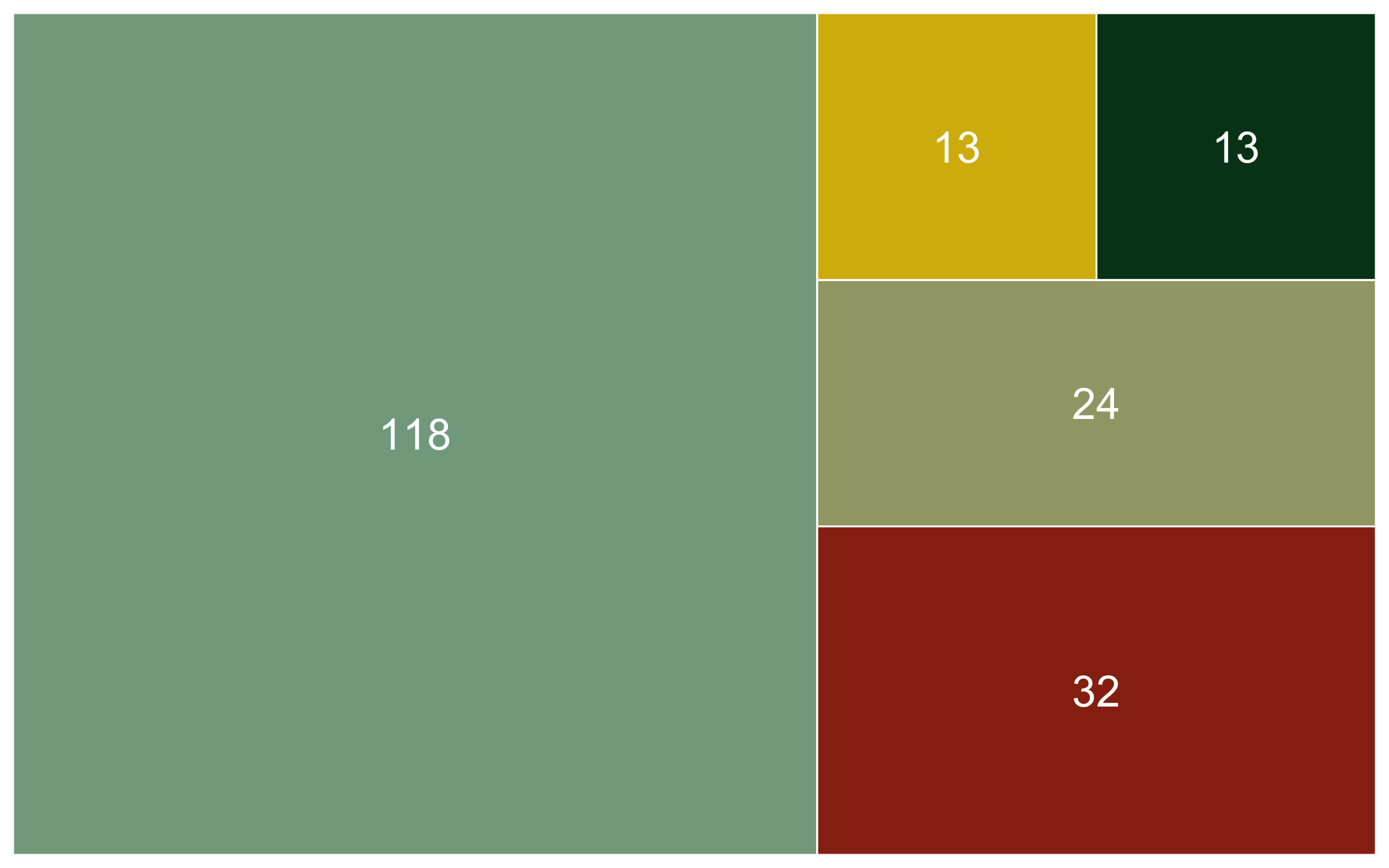We’ve covered spatial representation of the data (our map), as well as the kinds of species (the diet figures), now we can cover another dimention - time! We can make a timeline of the individual studies to see what time periods are best represented.

``````# Timeline
# Making the id variable a factor
# otherwise R thinks its a number
bird_models_traits\$id <- as.factor(as.character(bird_models_traits\$id))

(timeline_aus <- ggplot() +
geom_linerange(data = bird_models_traits, aes(ymin = minyear, ymax = maxyear,
colour = diet,
x = id),
size = 1) +
scale_colour_manual(values = wes_palette("Cavalcanti1")) +
labs(x = NULL, y = NULL) +
theme_bw() +
coord_flip())
``````

Well this looks untidy! The values are not sorted properly and it looks like a mess, but that happens often when making figures, part of the figure beautification journey. We can fix the graph with the code below.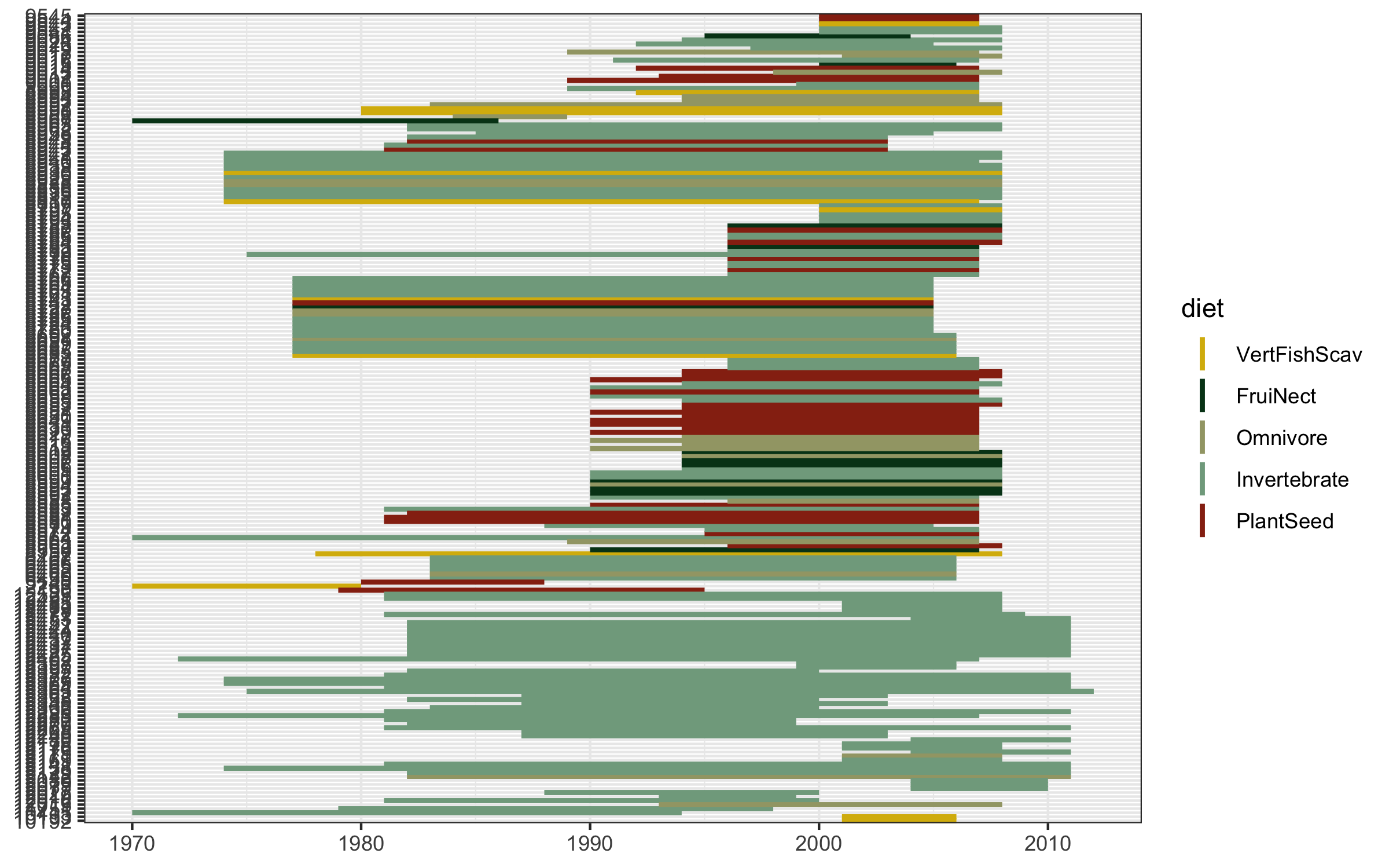``````# Create a sorting variable
bird_models_traits\$sort <- bird_models_traits\$diet
bird_models_traits\$sort <- factor(bird_models_traits\$sort, levels = c("VertFishScav",
"FruiNect",
"Omnivore",
"Invertebrate",
"PlantSeed"),
labels = c(1, 2, 3, 4, 5))

bird_models_traits\$sort <- paste0(bird_models_traits\$sort, bird_models_traits\$minyear)
bird_models_traits\$sort <- as.numeric(as.character(bird_models_traits\$sort))
``````

This sorting variable will help us arrange the studies first by species’ diet, then by when each study started.

``````(timeline_aus <- ggplot() +
geom_linerange(data = bird_models_traits, aes(ymin = minyear, ymax = maxyear,
colour = diet,
x = fct_reorder(id, desc(sort))),
size = 1) +
scale_colour_manual(values = wes_palette("Cavalcanti1")) +
labs(x = NULL, y = NULL) +
theme_bw() +
coord_flip() +
guides(colour = F) +
theme(panel.grid.minor = element_blank(),
panel.grid.major.y = element_blank(),
panel.grid.major.x = element_line(),
axis.ticks = element_blank(),
legend.position = "bottom",
panel.border = element_blank(),
legend.title = element_blank(),
axis.title.y = element_blank(),
axis.text.y = element_blank(),
axis.ticks.y = element_blank(),
plot.title = element_text(size = 20, vjust = 1, hjust = 0),
axis.text = element_text(size = 16),
axis.title = element_text(size = 20)))

ggsave(timeline_aus, filename = "timeline.png",
height = 5, width = 8)
``````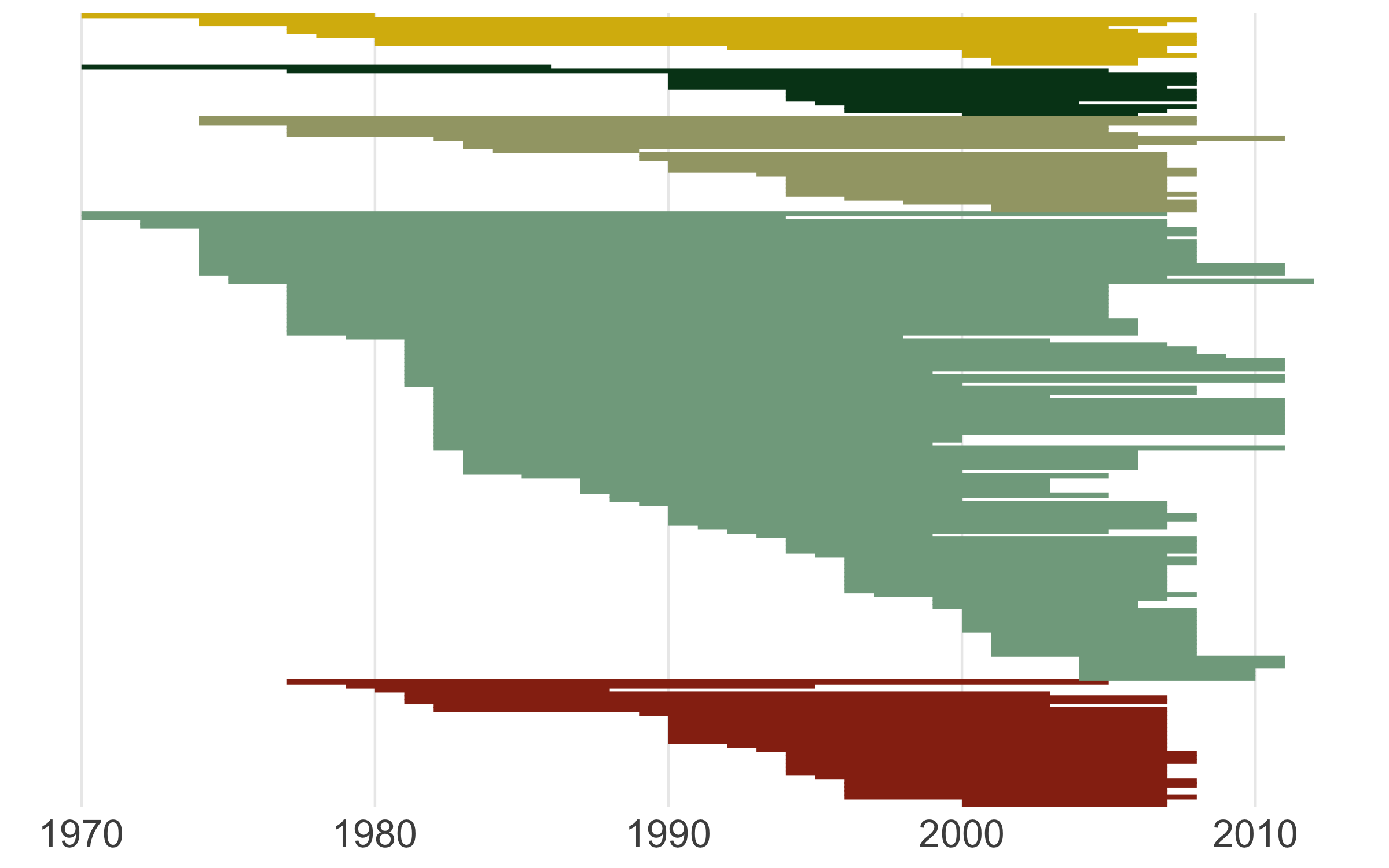For our final figure using our combined dataset of population trends and species’ traits, we will make a figure classic - the scatterplot. Body mass can sometimes be a good predictor of how population trends and extinction risk vary, so let’s find out if that’s true for the temporal changes in abundance across monitored populations of Australian birds.

``````# Combining the datasets
mass <- bird_traits %>% dplyr::select(species.name, BodyMass.Value) %>%
rename(mass = BodyMass.Value)
bird_models_mass <- left_join(aus_models, mass, by = "species.name") %>%
drop_na(mass)
``````

Now we’re ready to unwrap the data present (or if you’ve scrolled down, I guess it’s already unwrapped…). Whenever we are working with many data points, it can also be useful to “put a face (or a species) to the points”. For example, we can label some of the species at the extreme ends of the body mass spectrum.

``````(trends_mass <- ggplot(bird_models_mass, aes(x = log(mass), y = abs(estimate))) +
geom_point() +
geom_smooth(method = "lm") +
theme_clean() +
labs(x = "\nlog(mass)", y = "Absolute population change\n"))

# A more beautiful and clear version
(trends_mass <- ggplot(bird_models_mass, aes(x = log(mass), y = abs(estimate))) +
geom_point(colour = "turquoise4", size = 3, alpha = 0.3) +
geom_smooth(method = "lm", colour = "deepskyblue4", fill = "turquoise4") +
geom_label_repel(data = subset(bird_models_mass, log(mass) > 9),
aes(x = log(mass), y = abs(estimate),
label = common.name),
box.padding = 1, size = 5, nudge_x = 1,
# We are specifying the size of the labels and nudging the points so that they
# don't hide data points, along the x axis we are nudging by one
min.segment.length = 0, inherit.aes = FALSE) +
geom_label_repel(data = subset(bird_models_mass, log(mass) < 1.8),
aes(x = log(mass), y = abs(estimate),
label = common.name),
box.padding = 1, size = 5, nudge_x = 1,
min.segment.length = 0, inherit.aes = FALSE) +
theme_clean() +
labs(x = "\nlog(mass)", y = "Absolute population change\n"))

ggsave(trends_mass, filename = "trends_mass.png",
height = 5, width = 6)
``````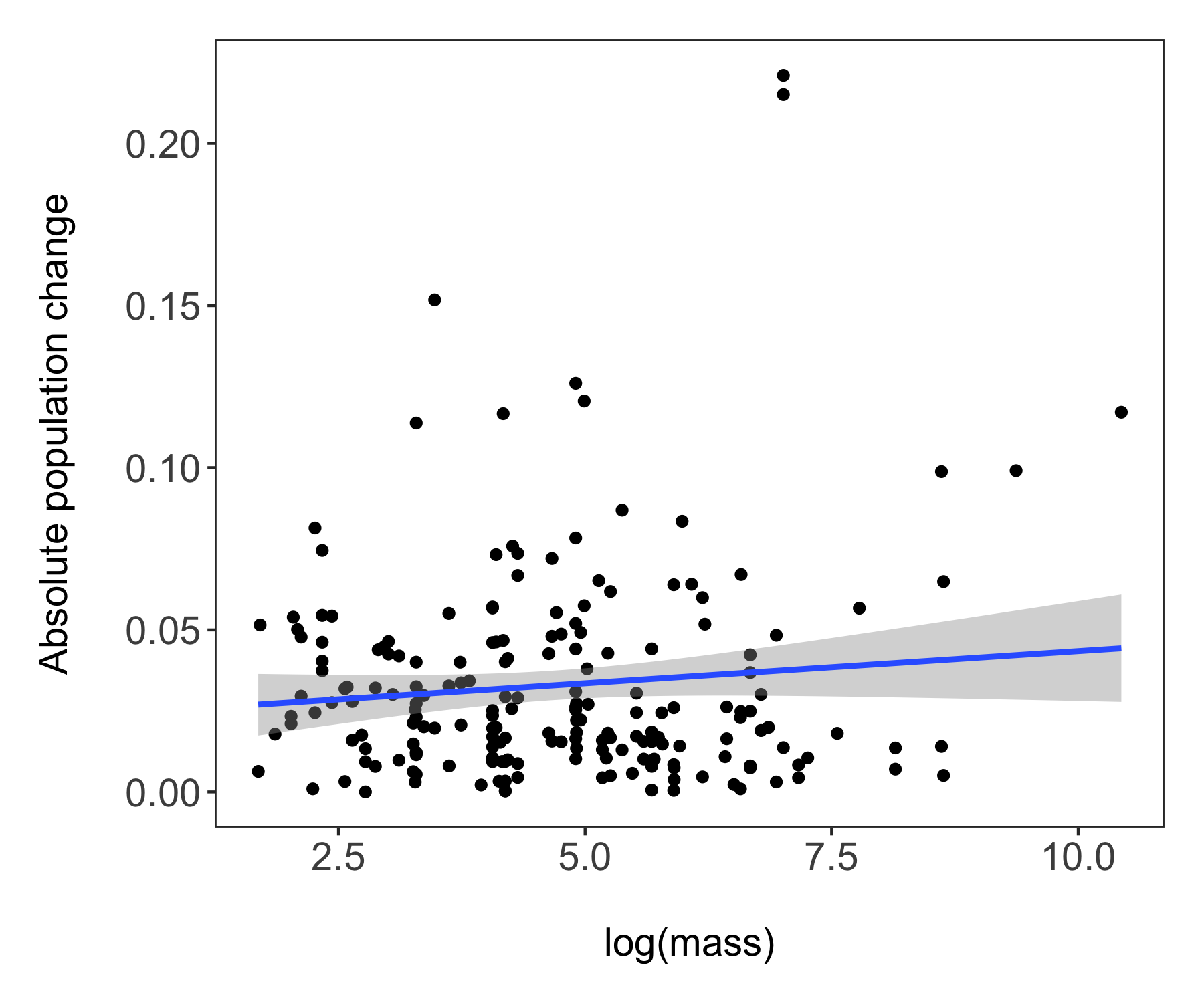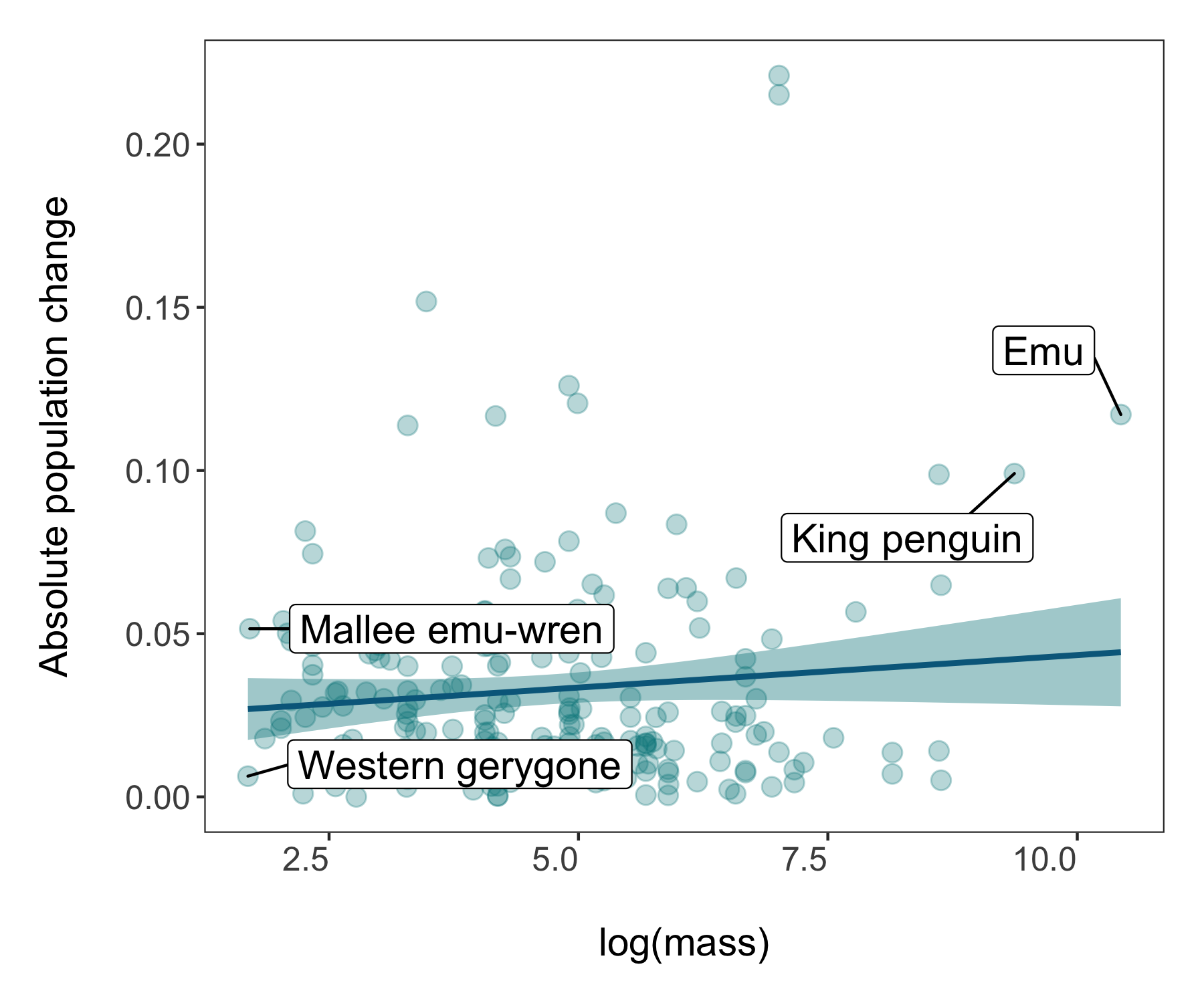### Congrats on taking three different types of figures on beautification journeys and all the best with the rest of your data syntheses!

If you’d like more inspiration and tips, check out the materials below!

## Extra resources

### You can also check out the package `patchwork` for another way to make multi-figure panels from `ggplot2` figures here.

To learn more about the power of pipes check out: the tidyverse website and the R for Data Science book.

To learn more about the `tidyverse` in general, check out Charlotte Wickham’s slides here.